https://doi.org/10.5194/gmd-15-8831-2022
https://doi.org/10.5194/gmd-15-8831-2022# Pathfinder v1.0.1: a Bayesian-inferred simple carbon–climate model to explore climate change scenarios

Thomas Bossy, Thomas Gasser, and Philippe Ciais
Abstract

The Pathfinder model was developed to fill a perceived gap within the range of existing simple climate models. Pathfinder is a compilation of existing formulations describing the climate and carbon cycle systems, chosen for their balance between mathematical simplicity and physical accuracy. The resulting model is simple enough to be used with Bayesian inference algorithms for calibration, which enables assimilation of the latest data from complex Earth system models and the IPCC sixth assessment report, as well as a yearly update based on observations of global temperature and atmospheric CO2. The model's simplicity also enables coupling with integrated assessment models and their optimization algorithms or running the model in a backward temperature-driven fashion. In spite of this simplicity, the model accurately reproduces behaviours and results from complex models – including several uncertainty ranges – when run following standardized diagnostic experiments. Pathfinder is an open-source model, and this is its first comprehensive description.

Share
Dates
1 Introduction

Simple climate models (SCMs) typically simulate global mean temperature change caused by either atmospheric concentration changes or anthropogenic emissions of CO2 and other climatically active species. They are most often composed of ad hoc parametric laws that emulate the behaviour of more complex Earth system models (ESMs). The emulation allows for simulating large ensembles of experiments that would be too costly to compute with ESMs. However, the SCM denomination refers to a fairly broad range of models whose complexity can go from a couple of boxes that only emulate one part of the climate system (e.g. a global temperature impulse response function; ) to hundreds of state variables representing the different cycles of greenhouse gases and their effect on climate change (e.g. the compact Earth system model OSCAR; ). Simpler models are easier and faster to solve, but they may not be adequate for all usages. Therefore, finding the “simplest but not simpler” model depends on a study's precise goals.

In our recent research, we have perceived a deficiency within the existing offer of SCMs, in spite of their large and growing number . We have therefore developed the Pathfinder model to fill this gap: it is a parsimonious CO2-only model that carefully balances simplicity and accuracy of representation of physical processes. Pathfinder was designed to fulfil three key requirements: (1) the capacity to be calibrated using Bayesian inference, (2) the capacity to be coupled with integrated assessment models (IAMs), and (3) the capacity to explore a very large number of climate scenarios to narrow down those compatible with limiting climate impacts. The latter motivated the model's name.

While these three requirements clearly call for the simplest model possible, as they all need a fast solving model, they also imply a certain degree of complexity. The Bayesian calibration requires an explicit representation of the processes (i.e. the variables) that are used to constrain the model. Coupling with IAMs requires accurately embedding the latest advances of climate sciences to be policy relevant . Exploring future climate impacts requires the flexibility to link additional (and potentially regional) impact variables to the core carbon–climate equations.

The Pathfinder model is essentially an integration of existing formulations, adapted to our modelling framework and goals. It is calibrated on Earth system models that contributed to the Coupled Model Intercomparison Project phase 6 (CMIP6), on additional data from the sixth assessment report of the IPCC (AR6), and on observations of global Earth properties up to the year 2021. The calibration philosophy of Pathfinder is to use complex models as prior information and only real-world observations and assessments combining many lines of evidence as constraints.

Compared to other SCMs , Pathfinder is much simpler than models like MAGICC , OSCAR , or even HECTOR . It is comparable in complexity to FaIR or BernSCM , although it is closer to the latter as it trades off an explicit representation of non-CO2 species for one of the carbon cycle's main components. This choice was made to help calibration, keep the model invertible, and make the model compatible with IAMs such as DICE (Nordhaus2017). While most SCMs are calibrated using procedures that resemble Bayesian inference , Pathfinder relies on an established algorithm whose implementation is fully tractable and that allows for an annual update as observations of atmospheric CO2 and global temperature become available.

Here, we present the first public release of Pathfinder and its source code. We first provide a detailed description of the model's equations. We then describe the Bayesian setup used for calibration, the sources of prior information for it, and the resulting posterior configuration. We end with a validation of the model using standard diagnostic simulations and quantitative metrics for the climate system and carbon cycle.

2 Equations

An overview of Pathfinder is presented in Fig. 1. The model is composed of a climate module, of three separate modules for the carbon cycle (ocean, land without land use and land permafrost), and of two additional modules describing global impacts: sea level rise (SLR) and surface ocean acidification. We do not emulate cycles of other non-CO2 gases. Mathematically, the model is driven by prescribing time series of any combination of two of four variables: global mean surface temperature (GMST) anomaly (T), global atmospheric CO2 concentration (C), global non-CO2 effective radiative forcing (Rx), and global anthropogenic emissions of CO2 (${E}_{{\mathrm{CO}}_{\mathrm{2}}}$). The model can therefore be run in the traditional emission-driven and concentration-driven modes but also in a temperature-driven mode (in terms of code, implemented as separate versions of the model). This is notably important for the calibration, during which it is driven by observations of GMST and atmospheric CO2.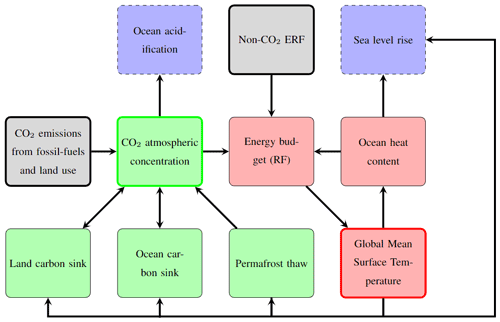Figure 1Pathfinder in a nutshell. Green blocks represent the carbon cycle, and red blocks represent the climate response. Blue blocks with dotted arrows are impacts that can be derived with the model. Grey blocks are variables that are directly related to anthropogenic activity. Possible inputs of the model are distinguishable through the bold contours of the blocks. In this scheme, arrows correspond to a forward mode where inputs would be ${E}_{{\mathrm{CO}}_{\mathrm{2}}}$ and Rx.

The following presents all equations of the models. Variables are noted using Roman letters and compiled in Tables B1 and B2. With a few exceptions, parameters are noted using Greek letters and are summarized in Tables B3 and B4. The model has 21 state variables that follow first-order differential equations in time. The time variable is denoted as t and kept implicit unless required.

## 2.1 Climate

The GMST change (T) induced by effective radiative forcing (ERF; R) is represented using a widely used two-box energy balance model with deep-ocean heat uptake efficacy . The first box represents the Earth surface's temperature (including atmosphere, land, and surface ocean), and the other one is the deep ocean's temperature (Td). Their time-differential equations are

$\begin{array}{}\text{(1)}& {\mathrm{\Theta }}_{\mathrm{s}}\phantom{\rule{0.33em}{0ex}}\frac{\mathrm{d}T}{\mathrm{d}t}=R-\frac{\mathit{\varphi }\phantom{\rule{0.33em}{0ex}}\mathrm{ln}\left(\mathrm{2}\right)}{{T}_{\mathrm{2}×}}\phantom{\rule{0.33em}{0ex}}T-{\mathit{ϵ}}_{\mathrm{heat}}\phantom{\rule{0.33em}{0ex}}\mathit{\theta }\phantom{\rule{0.33em}{0ex}}\left(T-{T}_{\mathrm{d}}\right),\end{array}$

and

$\begin{array}{}\text{(2)}& {\mathrm{\Theta }}_{\mathrm{d}}\phantom{\rule{0.33em}{0ex}}\frac{\mathrm{d}{T}_{\mathrm{d}}}{\mathrm{d}t}=\mathit{\theta }\phantom{\rule{0.33em}{0ex}}\left(T-{T}_{\mathrm{d}}\right),\end{array}$

where ϕ is the radiative parameter of CO2, T is the equilibrium climate sensitivity (ECS) at CO2 doubling, Θs is the heat capacity of the surface, Θd is the heat capacity of the deep ocean, θ is the heat exchange coefficient, and ϵheat is the deep-ocean heat uptake efficacy.

The global ERF is simply the sum of the CO2 contribution (${R}_{{\mathrm{CO}}_{\mathrm{2}}}$) from its change in atmospheric concentration (C), expressed using the IPCC AR5 formula , and that of non-CO2 climate forcers (Rx):

$\begin{array}{}\text{(3)}& R={R}_{{\mathrm{CO}}_{\mathrm{2}}}+{R}_{x},\end{array}$

with

$\begin{array}{}\text{(4)}& {R}_{{\mathrm{CO}}_{\mathrm{2}}}=\mathit{\varphi }\phantom{\rule{0.33em}{0ex}}\mathrm{ln}\phantom{\rule{-0.125em}{0ex}}\left(\frac{C}{{C}_{\mathrm{pi}}}\right),\end{array}$

where Cpi is the preindustrial atmospheric CO2 concentration.

The above energy balance model naturally provides the ocean heat content (OHC; Uohc) as

$\begin{array}{}\text{(5)}& {U}_{\mathrm{ohc}}={\mathit{\alpha }}_{\mathrm{ohc}}\phantom{\rule{0.33em}{0ex}}\left({\mathrm{\Theta }}_{\mathrm{s}}\phantom{\rule{0.33em}{0ex}}T+{\mathrm{\Theta }}_{\mathrm{d}}\phantom{\rule{0.33em}{0ex}}{T}_{\mathrm{d}}\right),\end{array}$

and the ocean heat uptake (OHU) as

$\begin{array}{}\text{(6)}& \frac{\mathrm{d}{U}_{\mathrm{ohc}}}{\mathrm{d}t}={\mathit{\alpha }}_{\mathrm{ohc}}\phantom{\rule{0.33em}{0ex}}\left({\mathrm{\Theta }}_{\mathrm{s}}\phantom{\rule{0.33em}{0ex}}\frac{\mathrm{d}T}{\mathrm{d}t}+{\mathrm{\Theta }}_{\mathrm{d}}\phantom{\rule{0.33em}{0ex}}\frac{\mathrm{d}{T}_{\mathrm{d}}}{\mathrm{d}t}\right),\end{array}$

where αohc is the fraction of energy used to warm the ocean (i.e. excluding the energy needed to heat up the atmosphere and land and to melt ice).

## 2.2 Sea level rise

Global SLR has been implemented in Pathfinder as a variable of interest to model climate change impacts. In this version, it is firstly a proof of concept, modelled in a simple yet sensible manner. The total sea level rise (Htot) is the sum of contributions from thermal expansion (Hthx), the Greenland ice sheet (GIS; Hgis), the Antarctic ice sheet (AIS; Hais), and glaciers (Hgla):

$\begin{array}{}\text{(7)}& {H}_{\mathrm{tot}}={H}_{\mathrm{thx}}+{H}_{\mathrm{gis}}+{H}_{\mathrm{ais}}+{H}_{\mathrm{gla}}.\end{array}$

The thermal expansion contribution scales linearly with the OHC :

$\begin{array}{}\text{(8)}& {H}_{\mathrm{thx}}={\mathrm{\Lambda }}_{\mathrm{thx}}\phantom{\rule{0.33em}{0ex}}{U}_{\mathrm{ohc}},\end{array}$

where Λthx is the scaling factor of the thermosteric contribution to SLR. Note, however, that the thermal capacity of the climate module does not match that of the real-world ocean , and so this equation cannot describe equilibrium SLR over millennial timescales.

To model contributions from ice sheets and glaciers, we followed the general approach of . The SLR caused by GIS follows a first-order differential equation with its specific timescale, and the equilibrium SLR from GIS is assumed to be a cubic function of GMST:

$\begin{array}{}\text{(9)}& \frac{\mathrm{d}{H}_{\mathrm{gis}}}{\mathrm{d}t}={\mathit{\lambda }}_{\mathrm{gis}}+\frac{\mathrm{1}}{{\mathit{\tau }}_{\mathrm{gis}}}\phantom{\rule{0.33em}{0ex}}\left({\mathrm{\Lambda }}_{\mathrm{gis}\mathrm{1}}\phantom{\rule{0.33em}{0ex}}T+{\mathrm{\Lambda }}_{\mathrm{gis}\mathrm{3}}\phantom{\rule{0.33em}{0ex}}{T}^{\mathrm{3}}-{H}_{\mathrm{gis}}\right),\end{array}$

where λgis is an offset parameter introduced because GIS was not in a steady state at the end of the preindustrial era, Λgis1 is the linear term of equilibrium of GIS SLR, Λgis3 is the cubic term of equilibrium of GIS SLR, and τgis is the timescale of the GIS contribution. The motivation for replacing the quadratic term of with a cubic one is the oddness of the cubic function that leads to negative (and not positive) SLR for negative T (which happens during the earlier years of the calibration run).

The contribution from glaciers is also a first-order differential equation with an equilibrium inspired by . We expanded it with a cubic term to account for the fact that we aggregate all glaciers together and allow more skewness in the curve describing the equilibrium SLR as a function of T. In addition, we added an exponential sensitivity to speed up the convergence to equilibrium under a warmer climate:

$\begin{array}{}\text{(10)}& \begin{array}{rl}\frac{\mathrm{d}{H}_{\mathrm{gla}}}{\mathrm{d}t}& ={\mathit{\lambda }}_{\mathrm{gla}}+\frac{\mathrm{exp}\phantom{\rule{-0.125em}{0ex}}\left({\mathit{\gamma }}_{\mathrm{gla}}\phantom{\rule{0.33em}{0ex}}T\right)}{{\mathit{\tau }}_{\mathrm{gla}}}\\ & \left({\mathrm{\Lambda }}_{\mathrm{gla}}\phantom{\rule{0.33em}{0ex}}\left(\mathrm{1}-\mathrm{exp}\phantom{\rule{-0.125em}{0ex}}\left(-{\mathrm{\Gamma }}_{\mathrm{gla}\mathrm{1}}\phantom{\rule{0.33em}{0ex}}T-{\mathrm{\Gamma }}_{\mathrm{gla}\mathrm{3}}\phantom{\rule{0.33em}{0ex}}{T}^{\mathrm{3}}\right)\right)-{H}_{\mathrm{gla}}\right),\end{array}\end{array}$

where λgla is an offset parameter accounting for the lack of initial steady state, Λgla is the SLR potential if all glaciers melted, Γgla1 is the linear sensitivity of glaciers' equilibrium to climate change, Γgla3 is the cubic sensitivity of glaciers' equilibrium to climate change, τgla is the timescale of the glaciers contribution, and γgla is the sensitivity of glaciers' timescale to climate change.

Following , the contribution from AIS is further divided into two terms, one for surface mass balance (SMB; Hais,smb) and one for solid ice discharge (SID; Hais,sid), so that ${H}_{\mathrm{ais}}={H}_{\mathrm{ais},\mathrm{smb}}+{H}_{\mathrm{ais},\mathrm{sid}}$. It is expected that precipitation will increase over Antarctica under higher GMST, leading to an increase in SMB and to a negative sea level rise contribution modelled as

$\begin{array}{}\text{(11)}& \frac{\mathrm{d}{H}_{\mathrm{ais},\mathrm{smb}}}{\mathrm{d}t}=-{\mathrm{\Lambda }}_{\mathrm{ais},\mathrm{smb}}\phantom{\rule{0.33em}{0ex}}T,\end{array}$

where Λais,smb is the AIS SMB sensitivity to climate change (expressed in sea level equivalent). At the same time, increasing surface ocean temperatures will cause more SID through basal melting, which we model using a first-order differential equation assumed to be independent of the SMB effect, and with a term that speeds up the effect the more SID happened:

$\begin{array}{}\text{(12)}& \frac{\mathrm{d}{H}_{\mathrm{ais},\mathrm{sid}}}{\mathrm{d}t}={\mathit{\lambda }}_{\mathrm{ais}}+\frac{\mathrm{1}+{\mathit{\alpha }}_{\mathrm{ais}}\phantom{\rule{0.33em}{0ex}}{H}_{\mathrm{ais},\mathrm{sid}}}{{\mathit{\tau }}_{\mathrm{ais}}}\phantom{\rule{0.33em}{0ex}}\left({\mathrm{\Lambda }}_{\mathrm{ais}}\phantom{\rule{0.33em}{0ex}}T-{H}_{\mathrm{ais},\mathrm{sid}}\right),\end{array}$

where λais is an offset parameter accounting for the lack of initial steady state, Λais is the SLR equilibrium of AIS SID, τais is the timescale of the AIS SID contribution, and αais is the sensitivity of the timescale to past SID. In the model's code, however, we directly solve for the total AIS contribution as

$\begin{array}{}\text{(13)}& \begin{array}{rl}\frac{\mathrm{d}{H}_{\mathrm{ais}}}{\mathrm{d}t}& =-{\mathrm{\Lambda }}_{\mathrm{ais},\mathrm{smb}}\phantom{\rule{0.33em}{0ex}}T+{\mathit{\lambda }}_{\mathrm{ais}}+\frac{\mathrm{1}+{\mathit{\alpha }}_{\mathrm{ais}}\phantom{\rule{0.33em}{0ex}}\left({H}_{\mathrm{ais}}-{H}_{\mathrm{ais},\mathrm{smb}}\right)}{{\mathit{\tau }}_{\mathrm{ais}}}\\ & \left({\mathrm{\Lambda }}_{\mathrm{ais}}\phantom{\rule{0.33em}{0ex}}T-\left({H}_{\mathrm{ais}}-{H}_{\mathrm{ais},\mathrm{smb}}\right)\right).\end{array}\end{array}$

## 2.3 Ocean carbon

To calculate the ocean carbon sink, we use the classic mixed-layer impulse response function model from , updated to the equivalent box model formulation of and extended in places to introduce parameter adjustments for calibration. In the model, the mixed layer is split into five boxes (subscript j), as represented in Fig. 2, so that the total carbon in the mixed-layer pool (Co) is

$\begin{array}{}\text{(14)}& {C}_{\mathrm{o}}=\sum _{j}{C}_{\mathrm{o},j}.\end{array}$

This total carbon mass is converted into a molar concentration of dissolved inorganic carbon (DIC; cdic) as follows:

$\begin{array}{}\text{(15)}& {c}_{\mathrm{dic}}=\frac{{\mathit{\alpha }}_{\mathrm{dic}}}{{\mathit{\beta }}_{\mathrm{dic}}}\phantom{\rule{0.33em}{0ex}}{C}_{\mathrm{o}},\end{array}$

where αdic is a fixed conversion factor and βdic is a scaling factor for the conversion. The latter can be seen as a factor multiplying the mixed-layer depth: it is 1 if the depth is unchanged from the original model.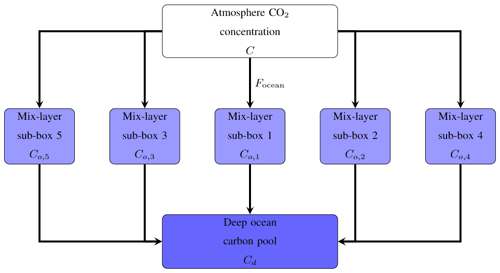Figure 2The ocean sink model in Pathfinder follows the structure of the mixed-layer pulse response function introduced by . The mixed layer is represented through five sub-pools that each have a different timescale for transport to the deep-ocean carbon pool.

The non-linear carbonate chemistry in the mixed layer is emulated in two steps. First, the model's original polynomial function is used to determine the partial pressure of CO2 affected by changes in DIC only (pdic):

$\begin{array}{}\text{(16)}& \begin{array}{rl}{p}_{\mathrm{dic}}=& \left(\mathrm{1.5568}-\mathrm{0.013993}\phantom{\rule{0.33em}{0ex}}{T}_{\mathrm{o}}\right)\phantom{\rule{0.33em}{0ex}}{c}_{\mathrm{dic}}\\ +& \left(\mathrm{7.4706}-\mathrm{0.20207}\phantom{\rule{0.33em}{0ex}}{T}_{\mathrm{o}}\right)\phantom{\rule{0.33em}{0ex}}{\mathrm{10}}^{-\mathrm{3}}\phantom{\rule{0.33em}{0ex}}{{c}_{\mathrm{dic}}}^{\mathrm{2}}\\ -& \left(\mathrm{1.2748}-\mathrm{0.12015}\phantom{\rule{0.33em}{0ex}}{T}_{\mathrm{o}}\right)\phantom{\rule{0.33em}{0ex}}{\mathrm{10}}^{-\mathrm{5}}\phantom{\rule{0.33em}{0ex}}{{c}_{\mathrm{dic}}}^{\mathrm{3}}\\ +& \left(\mathrm{2.4491}-\mathrm{0.12639}\phantom{\rule{0.33em}{0ex}}{T}_{\mathrm{o}}\right)\phantom{\rule{0.33em}{0ex}}{\mathrm{10}}^{-\mathrm{7}}\phantom{\rule{0.33em}{0ex}}{{c}_{\mathrm{dic}}}^{\mathrm{4}}\\ -& \left(\mathrm{1.5768}-\mathrm{0.15326}\phantom{\rule{0.33em}{0ex}}{T}_{\mathrm{o}}\right)\phantom{\rule{0.33em}{0ex}}{\mathrm{10}}^{-\mathrm{10}}\phantom{\rule{0.33em}{0ex}}{{c}_{\mathrm{dic}}}^{\mathrm{5}},\end{array}\end{array}$

where To is the preindustrial surface ocean temperature. Second, the actual partial pressure of CO2 (${p}_{{\mathrm{CO}}_{\mathrm{2}}}$) is calculated using an exponential climate sensitivity :

$\begin{array}{}\text{(17)}& {p}_{{\mathrm{CO}}_{\mathrm{2}}}=\left({p}_{\mathrm{dic}}+{C}_{\mathrm{pi}}\right)\phantom{\rule{0.33em}{0ex}}\mathrm{exp}\left({\mathit{\gamma }}_{\mathrm{dic}}\phantom{\rule{0.33em}{0ex}}T\right),\end{array}$

where γdic is the sensitivity of ${p}_{{\mathrm{CO}}_{\mathrm{2}}}$ to climate change.

The flux of carbon between the atmosphere and the ocean (Focean, defined positively if it is a carbon sink) is caused by the difference in partial pressure of CO2 in the atmosphere and at the oceanic surface, following an exchange rate that varies linearly with GMST, that is here used as a proxy for wind changes:

$\begin{array}{}\text{(18)}& {F}_{\mathrm{ocean}}={\mathit{\nu }}_{\mathrm{gx}}\phantom{\rule{0.33em}{0ex}}\left(\mathrm{1}+{\mathit{\gamma }}_{\mathrm{gx}}\phantom{\rule{0.33em}{0ex}}T\right)\phantom{\rule{0.33em}{0ex}}\left(C-{p}_{{\mathrm{CO}}_{\mathrm{2}}}\right),\end{array}$

where νgx is the preindustrial gas exchange rate and γgx is its sensitivity to climate change.

This flux of carbon entering the ocean is split between the mixed-layer carbon sub-pools, and this added carbon is subsequently transported towards the deep ocean at a rate specific to each sub-pool. This leads to the following differential equations:

$\begin{array}{}\text{(19)}& \frac{\mathrm{d}{C}_{\mathrm{o},j}}{\mathrm{d}t}=-\frac{{C}_{\mathrm{o},j}}{{\mathit{\kappa }}_{{\mathit{\tau }}_{\mathrm{o}}}\phantom{\rule{0.33em}{0ex}}{\mathit{\tau }}_{\mathrm{o},j}}+{\mathit{\alpha }}_{\mathrm{o},j}\phantom{\rule{0.33em}{0ex}}{F}_{\mathrm{ocean}},\phantom{\rule{1em}{0ex}}\forall j,\end{array}$

where αo,j are the sub-pools' splitting shares (with ${\sum }_{j}{\mathit{\alpha }}_{\mathrm{o},j}=\mathrm{1}$), τo,j are the sub-pools' timescales for transport to the deep ocean, and ${\mathit{\kappa }}_{{\mathit{\tau }}_{\mathrm{o}}}$ is a scaling factor applied to all sub-pools. Finally, the deep-ocean carbon pool (Cd) is obtained through mass balance:

$\begin{array}{}\text{(20)}& \frac{\mathrm{d}{C}_{\mathrm{d}}}{\mathrm{d}t}=\sum _{j}\frac{{C}_{\mathrm{o},j}}{{\mathit{\kappa }}_{{\mathit{\tau }}_{\mathrm{o}}}\phantom{\rule{0.33em}{0ex}}{\mathit{\tau }}_{\mathrm{o},j}}.\end{array}$

## 2.4 Ocean acidification

While in the real world, ocean acidification is directly related to the carbonate chemistry and the ocean uptake of anthropogenic carbon, we do not have a simple formulation at our disposal that could link it to our ocean carbon cycle module. We therefore use a readily available emulation of the surface ocean acidification (pH) that links it directly to the atmospheric concentration of CO2 with the following polynomial approximation:

where κpH is a scaling factor (that defaults to 1). We note that this approach is reasonable for the surface ocean, as it quickly equilibrates with the atmosphere (but it would not work for the deep ocean).

## 2.5 Land carbon

The land carbon module of Pathfinder is a simplified version of the one in OSCAR . It is shrunk down to four global carbon pools: vegetation, litter, and active and passive soil (see Fig. 3). All terrestrial biomes are lumped together, and there is therefore no accounting for the impact of land use change on the land carbon cycle in this version of Pathfinder. This is an extreme assumption – although very common in SCMs – motivated by simplicity, and it implies that CO2 emissions from fossil fuel burning and land use change are assumed to behave in the exact same way, in spite of their not doing so in reality .Figure 3The land sink model in Pathfinder is derived from OSCAR and represents the biosphere as a set of four carbon pools: vegetation, litter, and active and passive soil. These pools exchange carbon through fluxes whose direction is given by the arrows.

The vegetation carbon pool (Cv) results from the balance between net primary productivity (NPP; Fnpp), emission from wildfires (Efire), emission from harvest and grazing (Eharv), and loss of carbon from biomass mortality (Fmort):

$\begin{array}{}\text{(22)}& \frac{\mathrm{d}{C}_{\mathrm{v}}}{\mathrm{d}t}={F}_{\mathrm{npp}}-{E}_{\mathrm{fire}}-{E}_{\mathrm{harv}}-{F}_{\mathrm{mort}}.\end{array}$

NPP is expressed as its own preindustrial value multiplied by a function of CO2 and of GMST (rnpp). This function thus embeds the so-called CO2 fertilization effect, whereby NPP increases with atmospheric CO2, described using a generalized logarithmic functional form:

$\begin{array}{}\text{(23)}& {F}_{\mathrm{npp}}={F}_{\mathrm{npp}\mathrm{0}}\phantom{\rule{0.33em}{0ex}}{r}_{\mathrm{npp}},\end{array}$

with

$\begin{array}{}\text{(24)}& {r}_{\mathrm{npp}}=\left(\mathrm{1}+\frac{{\mathit{\beta }}_{\mathrm{npp}}}{{\mathit{\alpha }}_{\mathrm{npp}}}\phantom{\rule{0.33em}{0ex}}\left(\mathrm{1}-{\left(\frac{C}{{C}_{\mathrm{pi}}}\right)}^{-{\mathit{\alpha }}_{\mathrm{npp}}}\right)\right)\left(\mathrm{1}+{\mathit{\gamma }}_{\mathrm{npp}}\phantom{\rule{0.33em}{0ex}}T\right),\end{array}$

where Fnpp0 is the preindustrial NPP, βnpp is the CO2 fertilization sensitivity, αnpp is the CO2 fertilization shape parameter for saturation, and γnpp is the sensitivity of NPP to climate change (that can be positive or negative). The generalized logarithmic functional form implies that ${r}_{\mathrm{npp}}\to \left(\mathrm{1}+{\mathit{\beta }}_{\mathrm{npp}}\mathrm{ln}\left(C/{C}_{\mathrm{pi}}\right)\right)\left(\mathrm{1}+{\mathit{\gamma }}_{\mathrm{npp}}T\right)$ as ${\mathit{\alpha }}_{\mathrm{npp}}\to {\mathrm{0}}^{+}$.

Harvesting and mortality fluxes are taken proportional to the carbon pool itself even though in reality the mortality fluxes are climate dependent. For simplicity we assume a constant mortality following the equations in OSCAR :

$\begin{array}{}\text{(25)}& {E}_{\mathrm{harv}}={\mathit{\nu }}_{\mathrm{harv}}\phantom{\rule{0.33em}{0ex}}{C}_{\mathrm{v}},\end{array}$

and

$\begin{array}{}\text{(26)}& {F}_{\mathrm{mort}}={\mathit{\nu }}_{\mathrm{mort}}\phantom{\rule{0.33em}{0ex}}{C}_{\mathrm{v}},\end{array}$

where νharv is the harvesting or grazing rate and νmort is the mortality rate.

Wildfires emissions are also assumed to be proportional to the vegetation carbon pool, but with an additional linear dependency of the emission rate on CO2 (as a proxy of changes in leaf area index and evapotranspiration) and GMST (rfire):

$\begin{array}{}\text{(27)}& {E}_{\mathrm{fire}}={\mathit{\nu }}_{\mathrm{fire}}\phantom{\rule{0.33em}{0ex}}{r}_{\mathrm{fire}}\phantom{\rule{0.33em}{0ex}}{C}_{\mathrm{v}},\end{array}$

with

$\begin{array}{}\text{(28)}& {r}_{\mathrm{fire}}=\left(\mathrm{1}+{\mathit{\beta }}_{\mathrm{fire}}\left(\frac{C}{{C}_{\mathrm{pi}}}-\mathrm{1}\right)\right)\left(\mathrm{1}+{\mathit{\gamma }}_{\mathrm{fire}}\phantom{\rule{0.33em}{0ex}}T\right),\end{array}$

where νfire is the wildfire rate, βfire is the sensitivity of wildfires to CO2, and γfire is their sensitivity to climate change.

Soil carbon is divided into three pools. The litter carbon pool (Cs1) receives the mortality flux as sole input, it emits part of its carbon through heterotrophic respiration (Erh1), and it transfers another part to the next pool through stabilization (Fstab):

$\begin{array}{}\text{(29)}& \frac{\mathrm{d}{C}_{\mathrm{s}\mathrm{1}}}{\mathrm{d}t}={F}_{\mathrm{mort}}-{F}_{\mathrm{stab}}-{E}_{\mathrm{rh}\mathrm{1}}.\end{array}$

Similarly, the active soil carbon pool (Cs2) receives the stabilization flux, is respired (Erh2), and transfers carbon to the last pool through passivization (Fpass):

$\begin{array}{}\text{(30)}& \frac{\mathrm{d}{C}_{\mathrm{s}\mathrm{2}}}{\mathrm{d}t}={F}_{\mathrm{stab}}-{F}_{\mathrm{pass}}-{E}_{\mathrm{rh}\mathrm{2}}.\end{array}$

The passive carbon pool (Cs3) receives this final input flux and is respired (Erh3):

$\begin{array}{}\text{(31)}& \frac{\mathrm{d}{C}_{\mathrm{s}\mathrm{3}}}{\mathrm{d}t}={F}_{\mathrm{pass}}-{E}_{\mathrm{rh}\mathrm{3}}.\end{array}$

Although information pertaining to this fourth pool is not commonly provided by ESMs, it was introduced in Pathfinder to adjust the complex models' turnover time of soil carbon to better match isotopic data . For completeness, we note that the total heterotrophic respiration is ${E}_{\mathrm{rh}}={E}_{\mathrm{rh}\mathrm{1}}+{E}_{\mathrm{rh}\mathrm{2}}+{E}_{\mathrm{rh}\mathrm{3}}$, and the total soil carbon pool is ${C}_{\mathrm{s}}={C}_{\mathrm{s}\mathrm{1}}+{C}_{\mathrm{s}\mathrm{2}}+{C}_{\mathrm{s}\mathrm{3}}$.

All soil-originating fluxes are taken proportional to their pool of origin and multiplied by a function (rrh) explained hereafter. For the litter pool, this gives

$\begin{array}{}\text{(32)}& {E}_{\mathrm{rh}\mathrm{1}}={\mathit{\nu }}_{\mathrm{rh}\mathrm{1}}\phantom{\rule{0.33em}{0ex}}{r}_{\mathrm{rh}}\phantom{\rule{0.33em}{0ex}}{C}_{\mathrm{s}\mathrm{1}},\end{array}$

and

$\begin{array}{}\text{(33)}& {F}_{\mathrm{stab}}={\mathit{\nu }}_{\mathrm{stab}}\phantom{\rule{0.33em}{0ex}}{r}_{\mathrm{rh}}\phantom{\rule{0.33em}{0ex}}{C}_{\mathrm{s}\mathrm{1}},\end{array}$

where νrh1 is the litter respiration rate and νstab is the stabilization rate. For the active soil pool, we have

$\begin{array}{}\text{(34)}& {E}_{\mathrm{rh}\mathrm{2}}=\frac{{\mathit{\nu }}_{\mathrm{rh}\mathrm{23}}-{\mathit{\nu }}_{\mathrm{rh}\mathrm{3}}\phantom{\rule{0.33em}{0ex}}{\mathit{\alpha }}_{\mathrm{pass}}}{\mathrm{1}-{\mathit{\alpha }}_{\mathrm{pass}}}\phantom{\rule{0.33em}{0ex}}{r}_{\mathrm{rh}}\phantom{\rule{0.33em}{0ex}}{C}_{\mathrm{s}\mathrm{2}},\end{array}$

and

$\begin{array}{}\text{(35)}& {F}_{\mathrm{pass}}={\mathit{\nu }}_{\mathrm{rh}\mathrm{3}}\phantom{\rule{0.33em}{0ex}}\frac{{\mathit{\alpha }}_{\mathrm{pass}}}{\mathrm{1}-{\mathit{\alpha }}_{\mathrm{pass}}}\phantom{\rule{0.33em}{0ex}}{r}_{\mathrm{rh}}\phantom{\rule{0.33em}{0ex}}{C}_{\mathrm{s}\mathrm{2}},\end{array}$

and for the passive soil pool:

$\begin{array}{}\text{(36)}& {E}_{\mathrm{rh}\mathrm{3}}={\mathit{\nu }}_{\mathrm{rh}\mathrm{3}}\phantom{\rule{0.33em}{0ex}}{r}_{\mathrm{rh}}\phantom{\rule{0.33em}{0ex}}{C}_{\mathrm{s}\mathrm{3}},\end{array}$

where νrh23 is the soil respiration rate (averaged over active and passive pools), νrh3 is the passive soil respiration rate, and αpass is the fraction of passive carbon (over active plus passive soil carbon). This slightly convoluted formulation is motivated by the lack of information regarding the active/passive split in ESMs, which we alleviate using additional data during calibration.

In addition, the function rrh, describing the dependency of respiration (and related fluxes) on temperature and on the availability of fresh organic matter to be decomposed, is defined as follows:

$\begin{array}{}\text{(37)}& \begin{array}{rl}& {r}_{\mathrm{rh}}=\\ & \underset{\left(\mathrm{1}+{\mathit{\beta }}_{\mathrm{rh}}\left(\frac{{C}_{\mathrm{s}\mathrm{1}}}{{C}_{\mathrm{s}}}\frac{{C}_{\mathrm{s}}\left({t}_{\mathrm{0}}\right)}{{C}_{\mathrm{s}\mathrm{1}}\left({t}_{\mathrm{0}}\right)}-\mathrm{1}\right)\right)}{\underbrace{\left(\mathrm{1}+{\mathit{\beta }}_{\mathrm{rh}}\left(\frac{{C}_{\mathrm{s}\mathrm{1}}}{{C}_{\mathrm{s}\mathrm{1}}+{C}_{\mathrm{s}\mathrm{2}}+{C}_{\mathrm{s}\mathrm{3}}}\left(\mathrm{1}+\frac{{\mathit{\nu }}_{\mathrm{stab}}}{{\mathit{\nu }}_{\mathrm{rh}\mathrm{23}}}\right)-\mathrm{1}\right)\right)}}\mathrm{exp}\left({\mathit{\gamma }}_{\mathrm{rh}}\phantom{\rule{0.33em}{0ex}}T\right),\end{array}\end{array}$

where βrh is the sensitivity of the respiration to fresh organic matter availability (expressed here as the relative change in the ${C}_{\mathrm{s}\mathrm{1}}/{C}_{\mathrm{s}}$ ratio with regard to preindustrial times), and γrh is its sensitivity to climate change (equivalent to a “Q10” formulation with Q10=exp (10 γrh)).

Finally, the net carbon flux from the atmosphere to the land (Fland, defined positively if it is a carbon sink) is obtained as the net budget of all pools combined:

$\begin{array}{}\text{(38)}& {F}_{\mathrm{land}}={F}_{\mathrm{npp}}-{E}_{\mathrm{fire}}-{E}_{\mathrm{harv}}-{E}_{\mathrm{rh}},\end{array}$

and this system of equations leads to the following preindustrial steady state:

## 2.6 Permafrost carbon

As the land carbon cycle described in the previous section does not account for permafrost carbon, we implemented this feedback using the emulator developed by but aggregated into a unique global region. Figure 4 gives a representation of the permafrost module as described in the following. The emulation starts with a theoretical thawed fraction ($\overline{a}$) that represents the fraction of thawed carbon under steady-state for a certain level of local warming. It is formulated with a sigmoid function (that equals 0 at preindustrial and 1 under very high GMST):

$\begin{array}{}\text{(40)}& \begin{array}{rl}& \overline{a}=\\ & -{a}_{\mathrm{min}}+\frac{\left(\mathrm{1}+{a}_{\mathrm{min}}\right)}{{\left(\mathrm{1}+\left({\left(\mathrm{1}+\frac{\mathrm{1}}{{a}_{\mathrm{min}}}\right)}^{{\mathit{\kappa }}_{a}}-\mathrm{1}\right)\mathrm{exp}\left(-{\mathit{\gamma }}_{a}\phantom{\rule{0.33em}{0ex}}{\mathit{\kappa }}_{a}\phantom{\rule{0.33em}{0ex}}{\mathit{\alpha }}_{\mathrm{lst}}\phantom{\rule{0.33em}{0ex}}T\right)\right)}^{\frac{\mathrm{1}}{{\mathit{\kappa }}_{a}}}},\end{array}\end{array}$

where amin is the minimum thawed fraction (corresponding to 100 % frozen soil carbon), κa is a shape parameter determining the asymmetry of the function, γa is the sensitivity of the theoretical thawed fraction to local climate change, and αlst is the proportionality factor between local and global climate change.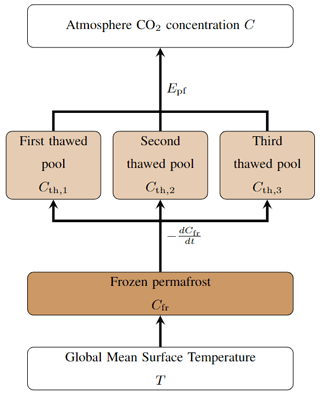Figure 4The permafrost carbon model in Pathfinder is taken from . The frozen pool dynamic lags behind a theoretical value that is determined by the temperature anomaly. Thawed carbon is then split between three pools that are emitted to the atmosphere at different rates.

The actual thawed fraction (a) then moves towards its theoretical value at a speed that depends on whether it is thawing (i.e. $a<\overline{a}$) or freezing (i.e. $a>\overline{a}$). This is written as a non-linear differential equation:

$\begin{array}{}\text{(41)}& \begin{array}{rl}\frac{\mathrm{d}a}{\mathrm{d}t}& =\mathrm{0.5}\phantom{\rule{0.33em}{0ex}}\left({\mathit{\nu }}_{\mathrm{thaw}}+{\mathit{\nu }}_{\mathrm{froz}}\right)\phantom{\rule{0.33em}{0ex}}\left(\overline{a}-a\right)\\ & +\mathrm{0.5}\phantom{\rule{0.33em}{0ex}}|\left({\mathit{\nu }}_{\mathrm{thaw}}-{\mathit{\nu }}_{\mathrm{froz}}\right)\phantom{\rule{0.33em}{0ex}}\left(\overline{a}-a\right)|,\end{array}\end{array}$

where νthaw is the rate of thawing and νfroz is the rate of freezing. Because νthaw>νfroz, the absolute value in the equation leads to the right-hand side being ${\mathit{\nu }}_{\mathrm{thaw}}\left(\overline{a}-a\right)$ if $a<\overline{a}$, or ${\mathit{\nu }}_{\mathrm{froz}}\left(\overline{a}-a\right)$ if $a>\overline{a}$. The change in the pool of frozen carbon (Cfr) naturally follows:

$\begin{array}{}\text{(42)}& \frac{\mathrm{d}{C}_{\mathrm{fr}}}{\mathrm{d}t}=-\frac{\mathrm{d}a}{\mathrm{d}t}\phantom{\rule{0.33em}{0ex}}{C}_{\mathrm{fr}\mathrm{0}},\end{array}$

where Cfr0 is the amount of frozen carbon at preindustrial times.

Thawed carbon is not directly emitted to the atmosphere: it is split into three thawed carbon sub-pools (Cth,j) that have their own decay time but are all affected by an additional function (rrt). This leads to the following budget equations:

$\begin{array}{}\text{(43)}& \frac{\mathrm{d}{C}_{\mathrm{th},j}}{\mathrm{d}t}=-{\mathit{\alpha }}_{\mathrm{th},j}\phantom{\rule{0.33em}{0ex}}\frac{\mathrm{d}{C}_{\mathrm{fr}}}{\mathrm{d}t}-\frac{{C}_{\mathrm{th},j}}{{\mathit{\kappa }}_{{\mathit{\tau }}_{\mathrm{th}}}\phantom{\rule{0.33em}{0ex}}{\mathit{\tau }}_{\mathrm{th},j}}\phantom{\rule{0.33em}{0ex}}{r}_{\mathrm{rt}},\phantom{\rule{1em}{0ex}}\forall j,\end{array}$

where αth,j is the sub-pools' splitting shares (with ${\sum }_{j}{\mathit{\alpha }}_{\mathrm{th},j}=\mathrm{1}$), τth,j is the sub-pools' decay times, and ${\mathit{\kappa }}_{{\mathit{\tau }}_{\mathrm{th}}}$ is a scaling factor applied to all sub-pools. The additional rrt function describes the sensitivity of heterotrophic respiration to climate change in boreal regions using a Gaussian formula:

$\begin{array}{}\text{(44)}& {r}_{\mathrm{rt}}=\mathrm{exp}\phantom{\rule{-0.125em}{0ex}}\left({\mathit{\kappa }}_{\mathrm{rt}}\phantom{\rule{0.33em}{0ex}}{\mathit{\gamma }}_{\mathrm{rt}\mathrm{1}}\phantom{\rule{0.33em}{0ex}}{\mathit{\alpha }}_{\mathrm{lst}}\phantom{\rule{0.33em}{0ex}}T-{\mathit{\kappa }}_{\mathrm{rt}}\phantom{\rule{0.33em}{0ex}}{\mathit{\gamma }}_{\mathrm{rt}\mathrm{2}}\phantom{\rule{0.33em}{0ex}}\left({\mathit{\alpha }}_{\mathrm{lst}}\phantom{\rule{0.33em}{0ex}}T{\right)}^{\mathrm{2}}\right),\end{array}$

where κrt is a factor scaling the sensitivity of thawed carbon against that of regular soil carbon, γrt1 is the sensitivity to local temperature change (i.e. a Q10), and γrt2 is the quadratic term in the latter sensitivity that represents a saturation effect. Noting that all the emitted carbon is assumed to be CO2, the global emission from permafrost (Epf) is thus

$\begin{array}{}\text{(45)}& {E}_{\mathrm{pf}}=\sum _{j}\frac{{C}_{\mathrm{th},j}}{{\mathit{\kappa }}_{{\mathit{\tau }}_{\mathrm{th}}}\phantom{\rule{0.33em}{0ex}}{\mathit{\tau }}_{\mathrm{th},j}}\phantom{\rule{0.33em}{0ex}}{r}_{\mathrm{rt}}.\end{array}$

## 2.7 Atmospheric CO2

The change in atmospheric concentration of CO2 is the budget of all carbon cycle fluxes to which we add the exogenous anthropogenic emissions (${E}_{{\mathrm{CO}}_{\mathrm{2}}}$):

$\begin{array}{}\text{(46)}& {\mathit{\alpha }}_{\mathrm{C}}\phantom{\rule{0.33em}{0ex}}\frac{\mathrm{d}C}{\mathrm{d}t}={E}_{{\mathrm{CO}}_{\mathrm{2}}}+{E}_{\mathrm{pf}}-{F}_{\mathrm{land}}-{F}_{\mathrm{ocean}},\end{array}$

where αC is the conversion factor from volume fraction to mass for CO2.

3 Bayesian calibration

## 3.1 Principle

Bayesian inference is a powerful tool for assimilating observational data into reduced-complexity models such as Pathfinder . The approach consists in deducing probability distributions of parameters from a priori knowledge on those distributions and on distributions of observations of some of the model's variables, using Bayes' theorem (Bayes1763). Summarily, the Bayesian calibration updates the joint distribution of parameters to make it as compatible with the constraints as possible given their prior estimates, which increases the internal coherence of Pathfinder by excluding combination of parameters that are unlikely.

Such a Bayesian calibration is vulnerable to the possibility that the priors draw on the same information as the constraints. However, given that Pathfinder is a patchwork of emulators whose parameters are obtained independently from one another and following differing experimental setups, we expect that the coherence of information contained within the priors and the constraints is very low. Our choice of using only complex models as prior information and only observations and assessments as constraints also aims at limiting this vulnerability.

Concretely, the posterior probability 𝒫post of a sample k from the joint parameters distribution ξk, conditional to a set of observations x, is proportional (symbol ) to its own prior probability 𝒫pre and to the likelihood of the model simulating x given ξk:

$\begin{array}{}\text{(47)}& {\mathcal{P}}_{\mathrm{post}}\left({\mathbit{\xi }}_{k}\mathrm{|}\mathbit{x}\right)\propto \mathcal{L}\left(\mathbit{x}\mathrm{|}{\mathbit{\xi }}_{k}\right)\phantom{\rule{0.33em}{0ex}}{\mathcal{P}}_{\mathrm{pre}}\left({\mathbit{\xi }}_{k}\right).\end{array}$

Here, we assume all observations are independently and identically distributed following a normal distribution (with mean values μx, and standard deviations σx expressed in real physical units), which leads to the following likelihood:

$\begin{array}{}\text{(48)}& \mathcal{L}\left(\mathbit{x}\mathrm{|}{\mathbit{\xi }}_{k}\right)=\prod _{i=\mathrm{1}}^{{n}_{x}}\frac{\mathrm{1}}{{\mathit{\sigma }}_{x,i}\phantom{\rule{0.33em}{0ex}}\sqrt{\mathrm{2}\mathit{\pi }}}\phantom{\rule{0.33em}{0ex}}\mathrm{exp}\phantom{\rule{-0.125em}{0ex}}\left(-\frac{\left({\mathcal{F}}_{i}\left({\mathbit{\xi }}_{k}\right)-{\mathit{\mu }}_{x,i}{\right)}^{\mathrm{2}}}{\mathrm{2}\phantom{\rule{0.33em}{0ex}}{\mathit{\sigma }}_{x,i}^{\mathrm{2}}}\right),\end{array}$

where i(ξk) is the model's output for the ith observable (out of nx) with input parameters ξk.

## 3.2 Implementation

The Pathfinder model is a set of differential equations with a number of input parameters, of which nξ are calibrated through Bayesian inference, and an additional two input variables provided as time series (i.e. one value per time step required). While the two input time series can be any combination of two out of four variables (anthropogenic CO2 emissions, non-CO2 ERF, atmospheric CO2 concentration, or GMST), for calibration we use the two most well-constrained variables that are direct physical observations of the global Earth system: atmospheric CO2 and GMST. These input time series cover the historical period from 1751 to 2020. Therefore, the ξk vector is

$\begin{array}{}\text{(49)}& {\mathbit{\xi }}_{k}={\left\{\mathit{\left\{}{\mathit{\xi }}_{j}{\mathit{\right\}}}_{j=\mathrm{1}}^{{n}_{\mathit{\xi }}},\mathit{\left\{}C\left(t\right){\mathit{\right\}}}_{t=\mathrm{1751}}^{\mathrm{2021}},\mathit{\left\{}T\left(t\right){\mathit{\right\}}}_{t=\mathrm{1751}}^{\mathrm{2021}}\right\}}_{k}.\end{array}$

However, to ease the computation by reducing the dimension of the system, we do not use annual time series of observations as inputs, but we assume that each input time series (for variable X being C or T) follows

$\begin{array}{}\text{(50)}& X\left(t\right)={X}_{\mathit{\mu }}\left(t\right)+{\stackrel{\mathrm{̃}}{\mathit{\sigma }}}_{X}\phantom{\rule{0.33em}{0ex}}{X}_{\mathit{\sigma }}\left(t\right)+{\mathit{ϵ}}_{X}\phantom{\rule{0.33em}{0ex}}\mathrm{AR}\mathrm{1}\left(t;{\mathit{\rho }}_{X}\right),\end{array}$

where Xμ and Xσ are fixed exogenous annual time series (i.e. structural parameters), ${\stackrel{\mathrm{̃}}{\mathit{\sigma }}}_{X}$ is the relative standard deviation of the time series (without noise), ϵX is the noise intensity, and AR1 is an autoregressive process of order 1 and autocorrelation parameter ρX. This assumption leads to the final expression of the ξk vector:

$\begin{array}{}\text{(51)}& {\mathbit{\xi }}_{k}={\left\{\mathit{\left\{}{\mathit{\xi }}_{j}{\mathit{\right\}}}_{j=\mathrm{1}}^{{n}_{\mathit{\xi }}},{\stackrel{\mathrm{̃}}{\mathit{\sigma }}}_{\mathrm{C}},{\mathit{ϵ}}_{\mathrm{C}},{\mathit{\rho }}_{\mathrm{C}},{\stackrel{\mathrm{̃}}{\mathit{\sigma }}}_{T},{\mathit{ϵ}}_{T},{\mathit{\rho }}_{T},\right\}}_{k}.\end{array}$

During this Bayesian assimilation, the Pathfinder model is run solely over the historical period (from 1750 to 2021), as the constraints concern only preindustrial or historical years. For the computation, the time-differential system of Pathfinder is solved using an implicit–explicit numerical scheme (also called IMEX), with a time step of a quarter of a year. This solving scheme relies on writing the differential equations of all state variables X as

$\begin{array}{}\text{(52)}& \frac{\mathrm{d}X}{\mathrm{d}t}=-\mathit{\nu }\phantom{\rule{0.33em}{0ex}}X+\mathcal{R},\end{array}$

where ν is the constant speed of the linear part of the differential equation and is its non-linear part, discretizing these equations as

$\begin{array}{}\text{(53)}& \frac{X\left(t+\mathit{\delta }t\right)-X\left(t\right)}{\mathit{\delta }t}=-\mathit{\nu }\phantom{\rule{0.33em}{0ex}}X\left(t+\mathit{\delta }t\right)+\mathcal{R}\left(t\right),\end{array}$

where δt is the solving time step (which is $\frac{\mathrm{1}}{{n}_{t}}$ times the annual time step of the model's inputs and outputs, here nt=4), and finally explicitly solving for all X(t+δt). We note this is also the default solving scheme for regular simulations with the model, although the value of nt can be altered and alternative schemes are available.

The Bayesian procedure itself is implemented using the Python computer language and specifically the PyMC3 package . The solving of Eq. (47) and its normalization are done using the package's full-rank automatic differentiation variational inference (ADVI) algorithm , with 100 000 iterations (and default algorithm options). The choice of variational inference instead of Markov chain Monte Carlo is motivated by the significant size of our model and the speed of ADVI. An additional strength of the full-rank version of the ADVI algorithm is its ability to generate correlated posterior distributions even if the prior ones are uncorrelated. Convergence of the algorithm was controlled through convergence of the ELBO metric . All results presented hereafter are obtained through drawing 2000 sets of parameters – which we call configurations – from the posterior or prior distributions.

## 3.3 Constraints

We use a set of 19 constraints related to all aspects of the model that correspond to the set of observations x in the Bayesian calibration. Many of the constraints are observations, but some are ranges assessed by expert panels such as the Global Carbon Project or the IPCC. They cover either a recent point in time or an assumed preindustrial equilibrium, and they are typically taken over a period of at least a few years to reduce the effect of natural variability.

Table 1 summarizes these constraints, the periods over which they are considered, and their distributions. The following subsections provide further details on the constraints, and the constraint distributions are shown in Fig. 6.

Table 1Constrained variables in Pathfinder, with values before and after calibration. Variables are noted under their text notation, and Tables B1 and B2 provide the corresponding notation in code. The uncertainty correspond to the 1σ uncertainty range.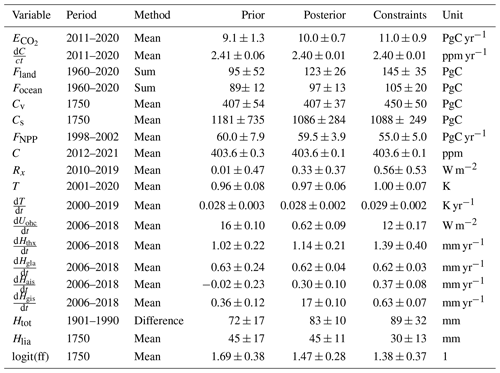### 3.3.1 Climate system

To constrain the temperature response, we use the same five data sets of observed GMST as in Sect. 3.4.8 to derive average and standard deviation of two constraints: the average GMST change and the average GMST yearly trend obtained through second-order accuracy gradient (Fornberg1988), both over the latest 20 years of data (2002–2021). Because these data sets are already used as input to the Bayesian setup, albeit in a different way, they do not provide much of a constraint and are used mostly to ensure that the ${\stackrel{\mathrm{̃}}{\mathit{\sigma }}}_{T}$ and ϵT parameters remain within sensible range.

To further constrain the climate system, we use the mean OHU assessed by the IPCC AR6 over 2006–2018 (Gulev et al.2021, Table 2.7) and the non-CO2 ERF (averaged over 2010-2019) also estimated for the AR6. The central value of the latter is taken from Dentener et al. (2021, Table AIII.3, and corresponding GitHub repository), and its uncertainty is constructed using data from Forster et al. (2021, Table 7.8) and assuming the ERF of all species are normally distributed and uncorrelated, but fully correlated in time for each separate species (which likely overestimates the uncertainty).

To better align with the IPCC AR6, we also constrain the ECS of our model (i.e. the T parameters). To do so, because the distribution of ECS cannot be assumed to be normal, we follow the framework of , who define the climate feedback factor ff so that ${T}_{\mathrm{2}×}={T}_{\mathrm{2}×}^{*}/\left(\mathrm{1}-\mathrm{ff}\right)$, where ${T}_{\mathrm{2}×}^{*}$ is the minimal ECS value (roughly corresponding to the Planck feedback). We assume this feedback factor follows a logit-normal distribution, which implies logit(ff) = $\mathrm{ln}\left(\mathrm{ff}/\left(\mathrm{1}-\mathrm{ff}\right)\right)$ = $\mathrm{ln}\left({T}_{\mathrm{2}×}/{T}_{\mathrm{2}×}^{*}-\mathrm{1}\right)$ follows a normal distribution. We therefore constrain logit(ff), using distribution parameters and a value of ${T}_{\mathrm{2}×}^{*}$ calibrated to fit the probabilistic ranges of ECS provided by the AR6. This fit of the ECS distribution is illustrated in Fig. B9.

### 3.3.2 Carbon cycle

Similarly to what is done with GMST, we constrain the atmospheric CO2 level over the latest 10 years of data (2012–2021) using the NOAA/ESRL data . The rest of the global CO2 budget is constrained using the 2021 Global Carbon Budget (GCB; ). We use the net atmospheric CO2 growth and total anthropogenic emissions (fossil and land use) over the last 10 years and the ocean and land carbon sinks accumulated since the beginning of the instrumental measurement period (1960–2020). Note that our definition of the land carbon sink ignoring land use change is consistent with that of the GCB.

Given its number of parameters and their inconsistent sources, we further constrain the land carbon module by considering present-day (mean over 1998–2002) NPP and preindustrial vegetation and soil carbon pools. These preindustrial pools are taken from the AR6 for the central value (Canadell et al.2021, Fig. 5.12), but their relative uncertainty is taken from the AR5 (Ciais et al.2013, Fig. 6.1) since it is lacking in the AR6. In addition, the soil carbon pool constraint is corrected downward by estimates of peatland carbon (Yu et al.2010, Table 1), since it is an ecosystem missing in TRENDY models (and in ours) but not in the IPCC assessments.

### 3.3.3 Sea level rise

To constrain the separate SLR contributions from thermal expansion, GIS, AIS, and glaciers, we use the model-based SLR speed estimates over the recent past (averaged over 2006–2018) reported in the AR6 (Fox-Kemper et al.2021, Table 9.5). To constrain the total contribution, we also use the historical (1901–1990) sea level rise inferred from tide gauges from the same source, although the value is corrected upward for the missed impact of uncharted glaciers .

Contrarily to all other modules, the SLR module is not assumed to start at steady state in 1750, which is represented through the λice ($\mathrm{ice}\in \left[\mathrm{gla},\mathrm{gis},\mathrm{ais}\right]$) parameters. We assume this is entirely due to the so-called Little Ice Age (LIA) relaxation, which we assume can be simply modelled in Pathfinder through exponential decay of our three ice-related contributions since t0=1750. This gives a net LIA contribution of ${H}_{\mathrm{lia}}={\sum }_{\mathrm{ice}}{\mathit{\lambda }}_{\mathrm{ice}}\phantom{\rule{0.33em}{0ex}}{\mathit{\tau }}_{\mathrm{ice}}\phantom{\rule{0.33em}{0ex}}\mathrm{exp}\phantom{\rule{-0.125em}{0ex}}\left(-\frac{t-{t}_{\mathrm{0}}}{{\mathit{\tau }}_{\mathrm{ice}}}\right)$. We constrain this diagnostic variable using the global SLR reported by over 1900–2005 for their control experiment.

## 3.4 Parameters (prior distributions)

Out of the model's 77 parameters, 33 are assumed to be fixed (i.e. they are structural parameters), and the remaining nξ=44 parameters are estimated through Bayesian inference. Prior distributions of the ξj parameters are assumed log-normal if the physical parameter must be defined positive, logit-normal if it must be between 0 and 1, and normal otherwise. To avoid extreme parameter values that could make the model diverge during calibration, the posterior distributions are bound to ${\mathit{\mu }}_{\mathit{\xi },j}±\mathrm{5}\phantom{\rule{0.33em}{0ex}}{\mathit{\sigma }}_{\mathit{\xi },j}$, where μξ,j and σξ,j are the mean and standard deviation of the jth parameter's prior distribution. These two values are taken from the literature, deduced from multi-model ensembles, or in a few instances arbitrarily set, as described in the following subsections. Note that when parameters are deduced from multi-model ensembles, there are effectively two rounds of calibration: first, a calibration on individual models using ordinary least-squares regressions to obtain prior distributions and, second, the Bayesian calibration itself that leads to the posterior distributions. In addition, the prior distributions of ${\stackrel{\mathrm{̃}}{\mathit{\sigma }}}_{X}$, ϵX and ρX are assumed normal, half-normal, and uniform, respectively. All prior distributions are assumed independent, meaning that the prior joint distribution ξ does not exhibit any covariance.

All parameters are summarized in Tables B5 and B6 along with their properties and values. The following subsections further explain how the prior distributions of the parameters are established, and these distributions are shown in Fig. 5.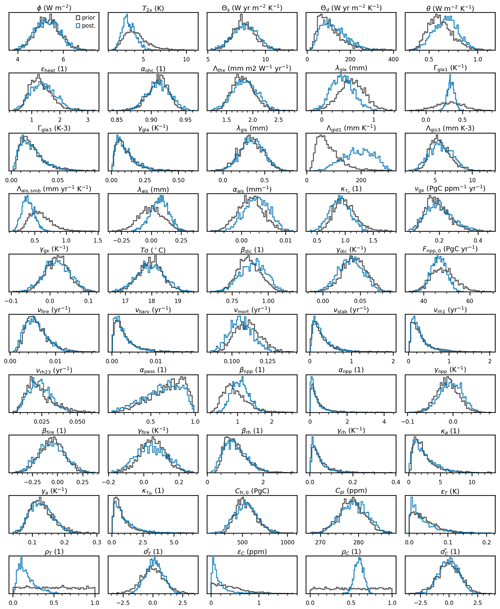Figure 5Parameter distributions before (black lines) and after (blue lines) the Bayesian calibration. Parameters are noted under their text notation, and Tables B3 and B4 provide the corresponding notation in code.

### 3.4.1 Climate

All the parameters of the climate module are calibrated. The prior distribution of the radiative parameter ϕ is taken from the AR5 (Myhre et al.2013, Table 8.SM.1). All other prior distributions of the parameters of the climate module (i.e. T, Θs, Θd, θ, and ϵheat) are taken from 35 CMIP6 models whose climate responses were derived for the AR6 using the “abrupt-4xCO2” experiment (Smith et al.2021, Sect. 7.SM.2.1, and corresponding GitHub repository). Here, T is simply assumed to be half the reported equilibrium temperature at quadrupled CO2. In addition, the prior distribution of the ocean warming fraction αohc is taken from the AR6 (Forster et al.2021, Table 7.1).

### 3.4.2 Sea level rise

Some parameters from the SLR module are structural: the maximum SLR contribution from glaciers (Λgla) is taken from Fox-Kemper et al. (2021, Sect. 9.6.3.2); the equilibrium AIS SLR (Λais) is from (Church et al.2013, Fig. 13.14); and the τgis, τgla, and τais timescales are the mean values from Mengel et al. (2016, Table S1) (assuming they provide the 90 % range of a log-normal distribution). All other parameters are calibrated. The prior distribution of the thermosteric parameter Λthx is taken from the AR6 (Fox-Kemper et al.2021, Sect. 9.2.4.1), as are the prior distributions of the preindustrial offset parameters λgis, λgla and λais (Fox-Kemper et al.2021, earliest period of Table 9.5). For the remaining parameters, we derive prior distributions using SLR projections compiled by for a number of ice sheets and glaciers models over various Representative Concentration Pathway (RCP) and Shared Socioeconomic Pathway (SSP) scenarios. Using the models' outputs, we apply Eq. (9) to estimate the Λgis1 and Λgis3 parameters; Eq. (10) for the Γgla1, Γgla3, and γgla parameters; and Eq. (12) for the Λais,smb and αais parameters. During these fits, all other parameters are assumed to take their default value if structural, and their best-guess value otherwise. Results of this calibration on the individual models compiled by are shown for each SLR contribution in Figs. B6, B7 and B8.

### 3.4.3 Ocean carbon

The ocean carbon cycle module has a number of structural parameters: αdic, all αo,j, and all τo,j are taken from Strassmann and Joos (2018, Tables A2 and A3, based on the Princeton model). The prior distribution of the adjustment factor ${\mathit{\kappa }}_{{\mathit{\tau }}_{\mathrm{o}}}$ is arbitrarily taken to apply a 20 % uncertainty on the oceanic transport timescales. All other prior distributions for this module's parameters are derived from 12 CMIP6 models with interactive carbon cycle that contributed to C4MIP . To is taken on average over the piControl simulation. νgx and γgx are calibrated by applying Eq. (18) to the models' outputs for the 1pctCO2, 1pctCO2-rad, and 1pctCO2-bgc experiments, while βdic and γdic are calibrated by applying Eqs. (14)–(17) and (19). Results of this calibration on the individual CMIP6 models are shown in Figs. B1 and B2.

### 3.4.4 Ocean acidification

In this version of Pathfinder, κpH is a structural parameter set to 1.

### 3.4.5 Land carbon

Parameters related to the passive soil carbon pool are taken from He et al. (2016, Table S5): νrh3 is structural, while αpass is not. All the prior distribution of the parameters related to the preindustrial steady state of the land carbon (i.e. Fnpp0, νfire, νharv, νmort, νstab, νrh1, and νcs) are derived from 11 TRENDYv7 models , exactly as for OSCAR v3.1 except that all biomes and regions are lumped together. The prior distribution of the remaining parameters are derived from 12 CMIP6 models that contributed to C4MIP . Using the models' outputs for the 1pctCO2, 1pctCO2-rad, and 1pctCO2-bgc experiments, we calibrated βnpp, αnpp, and γnpp through Eq. (24); βfire and γfire through Eq. (28); and βrh and γrh through Eq. (37). Results of this calibration on the individual CMIP6 models is shown in Figs. B3, B4, and B5.

### 3.4.6 Permafrost carbon

The permafrost module's parameters are recalibrated using the same algorithm as used by but adapted to the global formulation of Pathfinder. First, the algorithm is run once to obtain a set of parameters reproducing the behaviour of the global average of five permafrost models (with data from UVic added to the four original models). This gives the values of the structural parameters (i.e. αlst, γrt1, γrt2, κrt, amin, all αth,j, all τth,j, νthaw, and νfroz). Second, the algorithm is run five additional times for each of the five permafrost models separately, with the structural parameters established in the first step, to obtain prior distributions of the remaining parameters (i.e. Cfr0, κa, γa, and ${\mathit{\kappa }}_{{\mathit{\tau }}_{\mathrm{th}}}$).

### 3.4.7 Atmospheric CO2

The conversion factor αC is a structural parameter whose value is taken from the latest GCBs (e.g. Le Quéré et al.2018). The prior distribution of preindustrial CO2 concentration (Cpi) is taken from the AR6 (Gulev et al.2021, Sect.  2.2.3.2.1), assuming the difference between minimum and maximum over the 0–1850 period is representative of the 90 % uncertainty range.

### 3.4.8 Historical CO2 and GMST

The structural Xμ and Xσ time series are taken from the latest observations, as follows. Tμ and Tσ are taken as the average and standard deviation of five observational GMST data sets: HadCRUT5 , Berkeley Earth , GISTEMP , NOAAGlobalTemp , and JMA (2022). We use the 1850–1900 period to define our preindustrial baseline, and GMST change is assumed to be zero before the earliest date available in each data set. Regarding atmospheric CO2, Cμ is taken as the global value reported by NOAA/ESRL and Cσ as a constant ±1 ppm uncertainty for 1980 onward (this uncertainty is arbitrarily taken higher than the actual uncertainty estimated through instrumental measures to increase freedom in the calibration). Before that period, Cμ comes from the IPCC AR6 (Dentener et al.2021, Table AIII.1a), and Cσ is linearly interpolated backwards from the instrumental uncertainty in 1980 to the preindustrial one in 1750. Finally, the prior distribution of ρX is set to uniform over [0,1], that of ${\stackrel{\mathrm{̃}}{\mathit{\sigma }}}_{X}$ is a unit normal distribution, and that of ϵX is set arbitrarily to a half-normal of parameter 0.05 K for GMST and 0.5 ppm for CO2.

## 3.5 Results (posterior distributions)

The following subsections discuss the adjustments between the prior and posterior parameters that are the results of the Bayesian calibration, as well as the matching of the constraints. These sections constantly refer to Fig. 5 that shows the prior and posterior distributions of the model's parameters, Fig. 6 that shows those of the constrained variables, and Fig. 7 that displays the correlation matrix of the posterior parameters (there is no correlation among the prior parameters). Prior and posterior values of the parameters can also be retrieved from Table B6.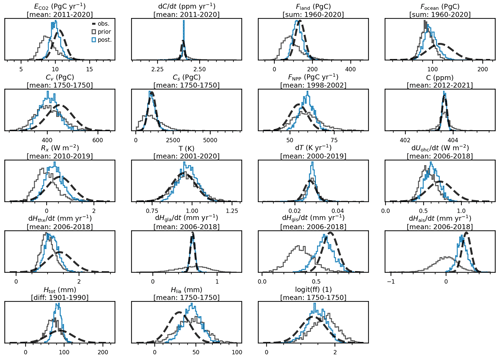Figure 6Distributions of the constrained variables. Dashed lines give the distributions used to constrain. Black lines give the distribution before calibration, while blue lines give the distribution posterior to calibration. Under a variable's name, we give the period over which the constraint is estimated and the data processing method: mean over the period, difference between last and first year, or sum of all the years over the period. (1750 is the preindustrial period). Variables are noted under their text notation, and Tables B1 and B2 provide the corresponding notation in code.

### 3.5.1 Climate system

Our climate-related constraints lead to adjusting all the parameters of the climate module. As explained in Sect. 3.4.8, the constraints for present-day GMST change and its derivative are met by construction.

The ECS (T) is the parameter with the strongest adjustment, since it is directly constrained. Its precise value is discussed hereafter in Sect. 4.2, but we note that it is unsurprisingly decreased, as the CMIP6 model ensemble tends to overestimate the ECS compared to the IPCC-assessed value. Consequently, our posterior logit(ff) matches the constraint well. The adjustment of the ECS significantly reduces the gap between our posterior distribution of the non-CO2 ERF and its constraint, although the posterior central value remains 41 % lower (but well within uncertainty range).

Among the dynamic parameters that are adjusted, we note that the deep-ocean heat capacity Θd is somewhat increased compared to the prior and that the heat exchange coefficient θ is also increased. These dynamic parameters are likely adjusted through our OHU constraint that is corrected in the posterior so the difference in the central values is lowered from 22 % to 14 %, which remains well within the uncertainty range.

Table 2Diagnostics of climate and carbon cycle responses in Pathfinder before and after Bayesian calibration. Comparison with AR6 and CMIP6 data is shown. For AR6 data we give the median and 90 % confidence interval, while for every other value we give the mean ±1σ.In addition, a number of weak but physically meaningful correlations across the climate module's parameters are found, such as a positive correlation between T and ϵheat (see, e.g. Geoffroy et al.2013a), a positive correlation between T and Θd (that tends to exclude configuration that would warm fast and high), and a negative correlation between T and ϕ (to match the GMST and ERF constraints together).

### 3.5.2 Carbon cycle

Similarly to GMST, the posterior distribution of atmospheric CO2 concentration matches the constraint by construction. Its derivative, however, is (slightly overly) corrected to match the GCB estimate. Global anthropogenic CO2 emissions are significantly increased to get closer to the GCB constraint, but their central value remains 9 % too low. Since these emissions are determined through mass balance and the atmospheric CO2 matches observations, this implies that the total carbon sinks (i.e. Fland+Focean) must be weaker.

This is confirmed for the ocean sink, as the posterior central value of Focean is 8 % lower than the constraint but still noticeably corrected if compared to the prior. This correction is explained by small adjustments in some parameters of the ocean carbon module. The mixed-layer depth is slightly increased through βdic. All other parameters remain mostly unaffected by the calibration, and only minor correlations are found. These results, along with the fact that our prior distribution spans only about half of the constraint's distribution, suggest that there is a structural limitation in our ocean carbon module that warrants further investigation.

It is also confirmed that the posterior land sink is weaker than the constraint, by 15 % for the central value, which is nevertheless a significant reduction of the prior gap of 34 %. To explain this adjustment, we observe that the CO2 fertilization sensitivities (βnpp and γnpp) are adjusted upwards. However, our constraint on present-day NPP prevents these adjustments from being too important, as the posterior distribution of this variable is similar to the prior and its central value remains 8 %–9 % higher than its constraint. An increased preindustrial NPP mechanically leads to an increase in preindustrial carbon pools, but these require further adjustments of the land carbon turnover rates, and most notably the mortality rate νmort and the passive carbon fraction αpass, to better match their constraints (of which the one on total soil carbon is perfectly met).

Table 3Comparison of SSP scenarios for GMST change projections (w.r.t. 1850–1900) to AR6 (Lee et al.2021, Table 4.5), and for ocean and land carbon storage projections to CMIP6 . Land carbon storage projections were corrected using the land use change emissions data from SSPs . For GMST data we give the median and the 90 % confidence interval while for every other values we give the mean ±1σ.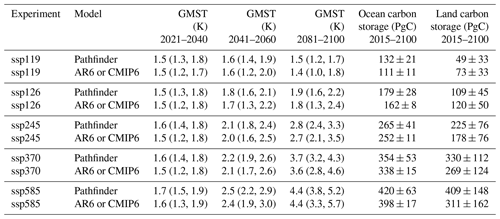Figure 7Correlation matrix of Pathfinder's parameters after the Bayesian calibration. Parameters are classified according to the equations they are related to, i.e. climate system, sea level, ocean carbon, land carbon, and permafrost carbon. Parameters are noted under their text notation, and Tables B3 and B4 provide the corresponding notation in code.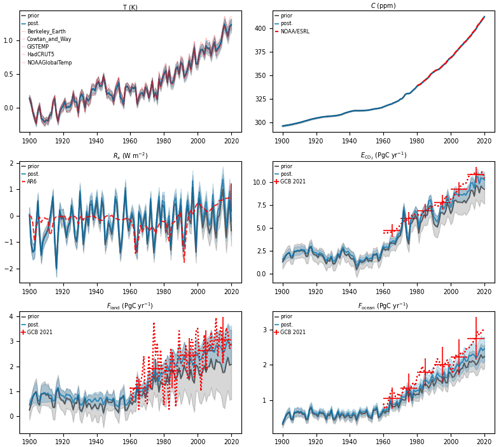Figure 8Historical time series of key variables from Pathfinder. Red lines are observations, black lines are the model's outputs before calibration, and blue lines are the same after calibration. Shaded areas and vertical bars correspond to the 1σ uncertainty range. Temperature observations are taken from HadCRUT5 , , Berkeley Earth , GISTEMP , and NOAA/MLOST . Other sources are NOAA/ESRL , GCB 2021 , and AR6 .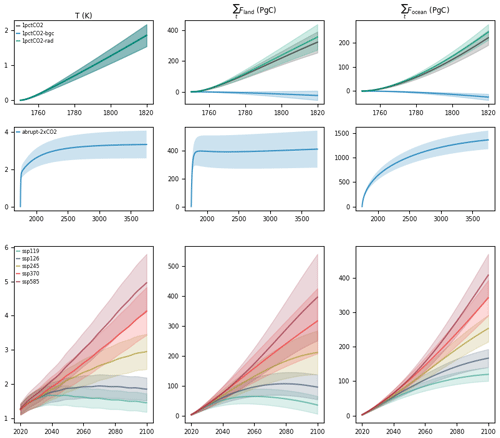Figure 9Time series of GMST change, integrated land carbon uptake and integrated ocean carbon uptake for idealized experiments (abrupt-2xCO2, 1pctCO2, 1pctCO2-bgc and 1pctCO2-rad) and projections according to SSP scenarios in Pathfinder. Shaded areas give the 1σ uncertainty range.

The land carbon module exhibits significant correlations among posterior parameters. This is likely a consequence of all the constraints combined as they dictate both the preindustrial steady state of the module and its transient response over the historical period. Eliminated configurations are those, for instance, that would show high initial carbon pools that are very sensitive to climate change (as these would lead to a very weak land sink) or that would exhibit a weak CO2 fertilization effect associated with a fast turnover time (that would also lead to a weak sink).

### 3.5.3 Sea level rise

The prior parameters of the SLR module are the least informed of our Bayesian setup. The model initially underestimates the thermal expansion, as well as the GIS and AIS SLR rates. The calibration brings the posterior distributions closer to their respective constraints but it always remains in the lower end of the uncertainty range. The correction is done by adjusting many of the module's parameters (most notably Λgis1, Λais,smb, λais or λgla) and by finding strong correlations among them (thus excluding physically unrealistic combinations).

The historical SLR is markedly corrected by the constraint: from a 19 % gap between the central values of the constraint and the prior estimate, to only 7 % after calibration. Here, we also note that the sum of individual contributions to historical SLR reported in AR6 do not match that total SLR (Fox-Kemper et al.2021, Table 9.5), which likely has some impact on the consistency between our constraints. Finally, although the LIA relaxation contribution is not altered by the calibration, as its central value remains 50 % too high, it is the likely source of the strong correlations found among the parameters of this module because it straightforwardly links the individual SLR contributions together.

4 Model diagnosis

## 4.1 Historical period

Because in the Bayesian setup we do not use annual time series of observations as constraints, the posterior distributions given in Fig. 6 do not inform on the whole dynamic of the model over the historical period. To further diagnose the model's behaviour, Fig. 8 gives the time series from 1900 to 2021 of six key variables. GMST and atmospheric CO2 match the historical observations very well via construction of these input time series. The non-CO2 ERF exhibits a very high variability, owing to our temperature-driven setup and the natural variability in the GMST input. Beyond that, the ERF time series is consistent with the AR6 estimates , albeit somewhat lower on average in the recent past, as seen with the posterior distribution. Consistently with the interpretation of carbon cycle variables in the calibration results, anthropogenic CO2 emissions, and the ocean and land carbon sinks are slightly underestimated compared to the GCB estimates . Several reasons could explain this discrepancy, from the lack of land use change in Pathfinder to the inconsistency of the GCB figures (that do not close the budget, while ours do). Nevertheless, the interest of the calibration is clearly illustrated, as the posterior uncertainty range covers observations much better than the prior one.

## 4.2 Idealized simulations

To complete the diagnosis of our model with common metrics used with climate and carbon models, we ran a set of standard idealized experiments, corresponding to the CMIP6 abrupt-2xCO2, 1pctCO2, 1pctCO2-bgc, and 1pctCO2-rad. A summary of these metrics' values is given in Table 2, and the resulting time series are shown in Fig. 9.

The abrupt-2xCO2 experiment sees an abrupt doubling of atmospheric CO2, and it is used to diagnose the model's ECS that is defined as the equilibrium temperature for a doubling of the preindustrial atmospheric concentration of CO2 (we acknowledge that it is superfluous with this version of Pathfinder since it is also a parameter). Using the GMST anomaly at the end of 1500 years of this experiment leads to an unconstrained estimate of ECS of 4.1 ± 1.3 K and a constrained estimate of 3.3 ± 0.7 K. Consistently, the latter value is between the ECS value extracted from CMIP6 models that is higher (3.7 ± 1.1 K) and the final value assessed in the AR6 that is lower (3.0 K, with a 67 % confidence interval between 2.5 and 4.0 K).

Using the 1pctCO2 experiment that sees a 1 % yearly increase in atmospheric CO2, we can estimate the model's transient climate response (TCR), which is defined as the GMST change after 70 years, when atmospheric concentration CO2 has just doubled. The CMIP6 models have a TCR of 2.0 ± 0.4 K . Pathfinder's unconstrained value is higher, at 2.2 ± 0.5 K, while the constrained one goes down to 1.9 ± 0.3 K. If we divide the TCR by the cumulative anthropogenic CO2 emissions compatible with the atmospheric CO2 increase in this experiment, we obtain an estimate of the transient climate response to emissions (TCRE). Similarly to the TCR, it is higher in the unconstrained ensemble and lower in the constrained one, when compared to CMIP6 models . Both downward adjustments of the TCR and TCRE are consistent with that of ECS, with the posterior TCRE matching the AR6 assessed range very well .

To look more closely at the carbon cycle, we perform two variants of the latter experiment: in 1pctCO2-rad, atmospheric CO2 only has a radiative effect, as it is kept at preindustrial level for the carbon cycle, whereas in 1pctCO2-bgc, atmospheric CO2 only has a biogeochemical effect, as the climate system sees only the preindustrial level. These three experiments are used to calculate the carbon–concentration (β) and carbon–climate (γ) feedback metrics that measure the carbon sinks' sensitivities to changes in atmospheric CO2 and GMST, respectively. We apply the same method as to calculate these, which leads to metrics at the time of CO2 doubling that are in line with CMIP6 models . As both carbon sinks were adjusted upwards by the Bayesian calibration, the constraints logically increased both βocean and βland, to values fairly close to those of the complex models. The γocean is not affected by the calibration and remains 45 % too low, which again suggests a structural limitation in our formulation of the ocean sink. This is, however, compensated for during calibration by the γland being 26 % higher than in complex models.

## 4.3 Scenarios

To further validate Pathfinder, we run the five SSP scenarios for which climate and carbon cycle projections were reported by a large enough number of models in the AR6 (namely, ssp119, ssp126, ssp245, ssp370, and ssp585). These simulations are run with prescribed CO2 concentration and non-CO2 ERF (the latter is taken from ). Time series of GMST and cumulative land and ocean sinks are shown in Fig. 9. Table 3 shows a comparison of the projected changes in GMST to the CMIP6 estimates (Lee et al.2021, Table 4.2) and of carbon pools to (since this was not directly reported in the AR6).

Be it on short-, mid- or long-term scales, Pathfinder's projections of GMST are very much in line with the one assessed by the IPCC in the AR6 based on multiple lines of evidence (Lee et al.2021, Table 4.5). The only significant difference is a smaller uncertainty range in our projections for the longer-term periods. Although this is the result of the efficiency of the Bayesian calibration, one might wonder whether the climate module is over-constrained (or equivalently, too limited in its number of parameters).

The ocean carbon storage appears to be overestimated by 5 % to 20 % by Pathfinder across SSP scenarios. This is consistent with the upward adjustment of the ocean carbon sink stemming from our Bayesian calibration. To compare the land carbon storage with CMIP6 models, because our land carbon module does not include land use change processes, we correct the value reported by complex models by the cumulative land use change emissions of each scenario . While the land carbon storage of Pathfinder is well in line under ssp126 (a scenario consistent with the 2 C target), it is underestimated in ssp119 (consistent with the 1.5 C target) and increasingly overestimated in higher warming scenarios. A likely explanation is that the climate–carbon feedback on land is underestimated in Pathfinder, as suggested by the γ metric seen in Sect. 4.2. Alternatively (or concurrently), the absence of loss of sink capacity caused by land cover change can explain the overestimation of the land sink under high CO2. The Pathfinder model's estimates of both sinks nonetheless remain well within the CMIP6 models' uncertainty ranges.

Our SLR emulator gives estimates (Table 4) that are always on the lower end of the range reported in the AR6 (Fox-Kemper et al.2021, Table 9.9). This can be explained by the fact that our individual SLR rate estimates are on the lower end of their respective constraints (see Sect. 3.5.3). This discrepancy also highlights potential structural limitations in the SLR module (e.g. too few separate contributions) and the difficulty of calibrating the module given the short time period of data available, both from complex models (that cover the 21st century only) and observations, compared to the long timescale of the SLR processes. Nevertheless, our estimates remain within the uncertainty range of the IPCC assessment, especially as we do not account for contribution from land water storage that causes an additional 0.03 [0.01, 0.04] m of SLR in all scenarios in 2100 .

Table 4Comparison of SSP scenarios between Pathfinder and AR6 for SLR (with respect to 1995–2014) and SLR speed projections (Fox-Kemper et al.2021, Table 9.9). We give the median value and the 90 % confidence interval in parentheses.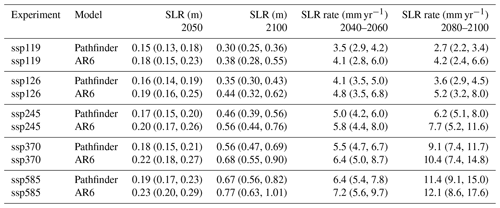5 Concluding remarks

In this paper, we have presented the Pathfinder model: a simple global carbon–climate model with selected impact variables, carefully designed to balance accuracy of representation and simplicity of formulation and calibrated through Bayesian inference on the latest data from Earth system models and observations. Pathfinder has been shown to perform very well in comparison to complex models, although there remains room for further improvement of the model and its calibration setup. We identify four main avenues to improve the model.

First, some parts of the model may well lean too much on the complexity side of the simplicity–accuracy balance we aimed to strike, owing to the creation process of Pathfinder that mostly compiled existing formulations. Future development should therefore strive to reduce complexity wherever possible. The ocean carbon sub-pools and perhaps the land carbon pools are potential leads in this respect.

Second, the ocean carbon module alone appears to be limited by its structure, which has been inherited from a 25-year-old (yet seminal) article . Although it is undoubtedly a significant undertaking, developing an alternative formulation of the ocean carbon dynamic, calibrated on state-of-the-art ocean models and properly connected to ocean pH and the ocean of the climate module, would benefit more than just the SCM community.

Third, integration of land use and land cover change in such a model appears warranted, despite the difficulty of doing so in a physically sensible yet simple manner. Given our expertise with the OSCAR model and its bookkeeping module , we are confident that this can be done, although it will demand extra care to keep the model compatible with the IAMs it is also meant to be linked to.

Fourth, the Bayesian setup can be extended, notably by including more time periods for the existing constraints but also by introducing and constraining entirely new variables such as isotopic ratios or inter-hemispheric gradients ; however, a balance must be struck with respect to the calibration's computation time. Here, we caution against including complex models' results as constraints in the Bayesian calibration, as was done for the IPCC AR6 , as it goes against the philosophy of Pathfinder to use complex models' results as prior information only.

Fifth, although our model is restricted to CO2 by design because of how IAMs like DICE (Nordhaus2017) are also limited to CO2 emissions, we can imagine many reasons why one would want to add non-CO2 climate forcers into Pathfinder. We would suggest doing so by following the model's philosophy: that is, by taking existing reduced-complexity formulations such as something between FaIR and OSCAR and adding the new parameters into the Bayesian setup with the relevant observational constraints.

In spite of these few shortcomings and potential development leads, Pathfinder v1.0.1 is a powerful tool that fits the niche it has been created for perfectly. We will further demonstrate the strengths and flexibility of Pathfinder in other publications. Meanwhile, we invite the community to seize this open-source model and use it in any study that could benefit from a simple, fast, and accurate carbon–climate model aligned with the latest climate science.

## A1 Technical requirements

Pathfinder has been developed and run in Python (v3.7.6) (https://docs.python.org/3.7/, last access: 5 December 2022), preferentially using IPython (v7.19.0) . Currently, packages required to run it are NumPy (v1.19.2) , SciPy (v1.5.2) , and Xarray (v0.16.0) , and it has hard-coded dependencies on PyMC3 (v3.8) and Theano (v1.0.4) that are in fact used only for calibration. Other versions of Python or these packages were not tested.

The calibration procedure takes about 9 h to run on a desktop computer (with a base speed of 3.4 GHz). Simple use of the model is much faster: the idealized experiments and SSP scenarios for this description paper, which represent 2984 simulated years, were run in about 20 min for all 2000 configurations and on a single core. A single simulated year takes a few tenths of a second, although a number of options in the model can drastically alter this performance. Note also that this scales sub-linearly with the amount of configurations or scenarios because of the internal workings of the Xarray package, albeit at the cost of increased demand in random-access memory.

## A2 Known issues

Two relatively benign issues that have been identified during development remain unsolved. First, the model requires a high number of sub-time steps (i.e. high nt) to remain stable under high CO2 because of the ocean carbon cycle. Second, the version of the model that is driven by T and Rx time series is extremely sensitive to its inputs because it mathematically requires the first two derivatives of T and the first derivative of Rx.

## A3 Changelog

Brief description of the successive versions of Pathfinder.

v1.0.1.

Exact same physical equations and numerical values as v1.0. Added best-guess parameters calculated as the average of the posterior distribution, and corresponding historical outputs, for single-configuration runs. Improved README and MANUAL files.

v1.0.

First release. Exact model described in the preprint version of this paper .

Appendix B: Additional figures and tables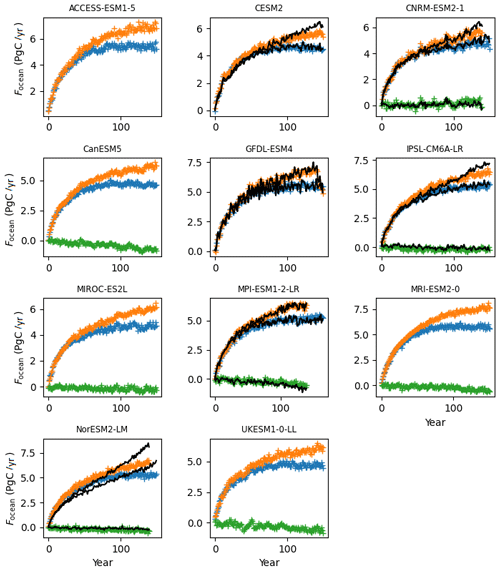Figure B1Calibration to estimate prior νgx and γgx from CMIP6 time series of Focean. We fit our equation on the results of the +1 % CO2 (1pctCO2) experiment (in blue) and its variants 1pctCO2-rad (in green) and 1pctCO2-bgc (in orange). Coloured markers are CMIP6 model data, while the solid black lines show the fit from Pathfinder. Panels without a black line indicate that at least one of the required variables was not reported by the complex model.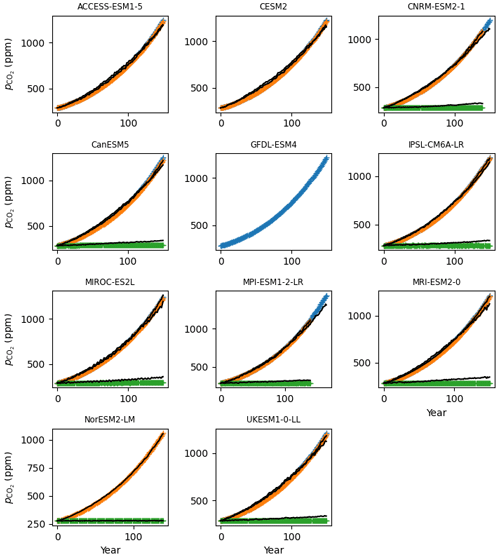Figure B2Calibration to estimate prior βdic and γdic from CMIP6 time series of ${p}_{{\mathrm{CO}}_{\mathrm{2}}}$. We fit our equation on the results of the +1 % CO2 (1pctCO2) experiment (in blue) and its variants 1pctCO2-rad (in green) and 1pctCO2-bgc (in orange). Coloured markers are CMIP6 model data, while the solid black lines show the fit from Pathfinder. Panels without a black line indicate that at least one of the required variables was not reported by the complex model.Figure B3Calibration to estimate prior βef and γef from CMIP6 time series of rfire, shown as a ratio over its preindustrial value. We fit our equation on the results of the +1 % CO2 (1pctCO2) experiment (in blue) and its variants 1pctCO2-rad (in green) and 1pctCO2-bgc (in orange). Coloured markers are CMIP6 model data, while the solid black lines show the fit from Pathfinder. Panels without a black line indicate that at least one of the required variables was not reported by the complex model.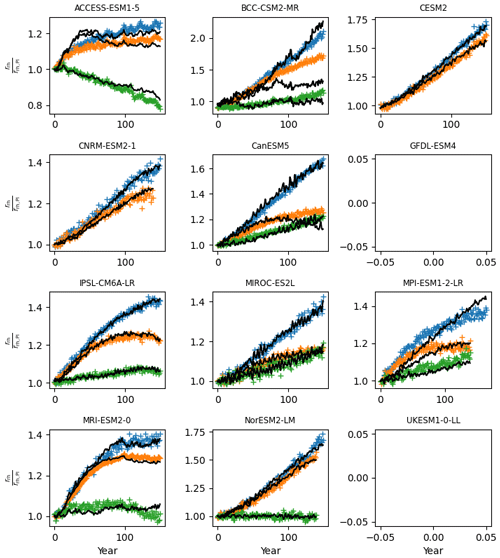Figure B4Calibration to estimate prior βrh and γrh from CMIP6 time series of rrh, shown as a ratio over its preindustrial value. We fit our equation on the results of the +1 % CO2 (1pctCO2) experiment (in blue) and its variants 1pctCO2-rad (in green) and 1pctCO2-bgc (in orange). Coloured markers are CMIP6 model data, while the solid black lines show the fit from Pathfinder. Panels without a black line indicate that at least one of the required variables was not reported by the complex model.Figure B5Calibration to estimate prior βnpp, αnpp, and γnpp from CMIP6 time series of rnpp, shown as a ratio over its preindustrial value. We fit our equation on the results of the +1 % CO2 (1pctCO2) experiment (in blue) and its variants 1pctCO2-rad (in green) and 1pctCO2-bgc (in orange). Coloured markers are CMIP6 model data, while the solid black lines show the fit from Pathfinder. Panels without a black line indicate that at least one of the required variables was not reported by the complex model.Figure B6Calibration to estimate the prior of GIS SLR module parameters (Λgis1, Λgis3, and λgis0). We fit our equation on the compiled outputs from for which there is more than one scenario available. Each panel's title displays the name of the institute, model, and configuration used. Coloured markers are the model data, while the solid black lines show the fit from Pathfinder.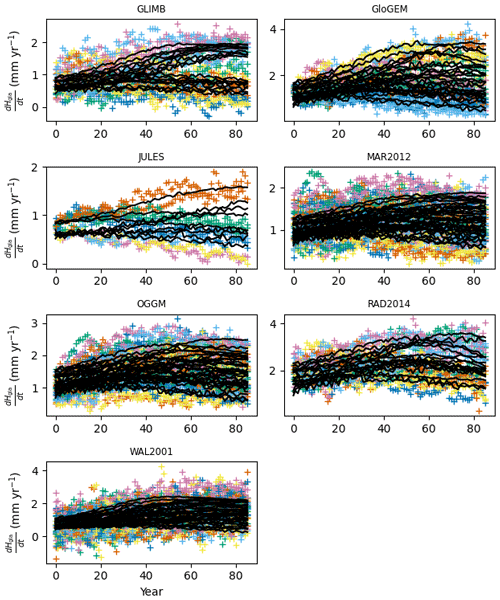Figure B7Calibration to estimate the prior of glacier SLR module parameters (Γgla1, Γgla3, γgla, and λgla0). We fit our equation on the compiled outputs from for which there is more than one scenario available. Each panel's title displays the name of the model or study used. Coloured markers are the model data, while the solid black lines show the fit from Pathfinder.Figure B8Calibration to estimate the prior of AIS SLR module parameters (Λais,smb, αais, and λais0). We fit our equation on the compiled outputs from for which there is more than one scenario available. Each panel's title displays the name of the institute, model and configuration used. Coloured markers are the models data while the solid black lines show the fit from Pathfinder.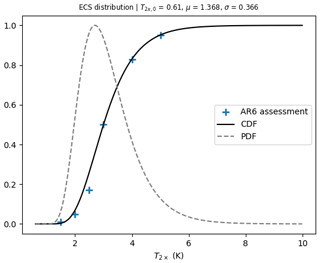Figure B9Distribution of the logit of the ECS (T) inferred from AR6. Blue points are the assessments from AR6, the solid line is the cumulative distributive function (CDF) fitted on those assessments, and the dashed line is the PDF associated with the CDF (arbitrary scale). The value of the fitted parameters is given above the plot.

Table B1Summary of Pathfinder's equation variables in climate, sea level, and ocean carbon modules.Table B2Summary of Pathfinder's equation variables for land carbon, permafrost, and atmospheric modules.Table B4Parameters used in the permafrost, land carbon modules, and for calibration.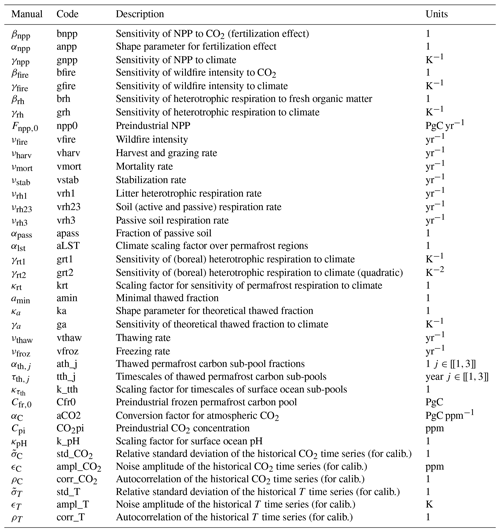Table B5Structural parameter values. Parameters are noted under their code notation, and Tables B3 and B4 provide the corresponding notation in text.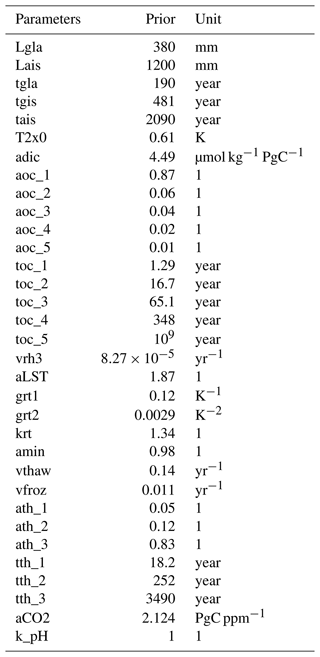Table B6Calibrated parameter values before and after Bayesian calibration. Parameters are noted under their code notation, and Tables B3 and B4 provide the corresponding notation in text. The uncertainty corresponds to the 1σ uncertainty range.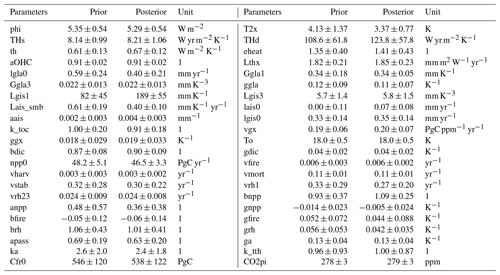Code and data availability

The source code of Pathfinder is openly available at https://github.com/tgasser/Pathfinder (last access: 5 December 2022). A frozen version of the code and data as developed in the paper can be found on Zenodo at https://doi.org/10.5281/zenodo.7003848 (Gasser2022).

Author contributions

TG developed the model, with contributions from TB regarding sea level rise and PC regarding land carbon cycle. TG coded the model and its calibration. TB ran the diagnostic simulations and made the final figures. TB and TG wrote the manuscript with input from PC.

Competing interests

The contact author has declared that none of the authors has any competing interests.

Disclaimer

Publisher's note: Copernicus Publications remains neutral with regard to jurisdictional claims in published maps and institutional affiliations.

Acknowledgements

We thank Andrew H. MacDougall for sharing permafrost simulations made with UVic, and Côme Cheritel for the first version of Figs. 1–4.

Financial support

Development of Pathfinder was supported by the European Union's Horizon 2020 research and innovation programme under grant agreement no. 820829 (CONSTRAIN project) and by the Austrian Science Fund (FWF) under grant agreement no. P31796-N29 (ERM project).

Review statement

This paper was edited by Marko Scholze and reviewed by Ian Enting and one anonymous referee.

References

Armour, K. C.: Energy budget constraints on climate sensitivity in light of inconstant climate feedbacks, Nat. Clim. Change, 7, 331–335, 2017. a

Arora, V. K., Katavouta, A., Williams, R. G., Jones, C. D., Brovkin, V., Friedlingstein, P., Schwinger, J., Bopp, L., Boucher, O., Cadule, P., Chamberlain, M. A., Christian, J. R., Delire, C., Fisher, R. A., Hajima, T., Ilyina, T., Joetzjer, E., Kawamiya, M., Koven, C. D., Krasting, J. P., Law, R. M., Lawrence, D. M., Lenton, A., Lindsay, K., Pongratz, J., Raddatz, T., Séférian, R., Tachiiri, K., Tjiputra, J. F., Wiltshire, A., Wu, T., and Ziehn, T.: Carbon–concentration and carbon–climate feedbacks in CMIP6 models and their comparison to CMIP5 models, Biogeosciences, 17, 4173–4222, https://doi.org/10.5194/bg-17-4173-2020, 2020. a, b, c, d, e, f

Bayes, T.: LII. An essay towards solving a problem in the doctrine of chances. By the late Rev. Mr. Bayes, FRS communicated by Mr. Price, in a letter to John Canton, AMFR S, Philos. T. Roy. Soc. Lond., 53, 370–418, 1763. a

Bernie, D., Lowe, J., Tyrrell, T., and Legge, O.: Influence of mitigation policy on ocean acidification, Geophys. Res. Lett., 37, 1–5, https://doi.org/10.1029/2010GL043181, 2010. a

Blei, D. M., Kucukelbir, A., and McAuliffe, J. D.: Variational inference: A review for statisticians, J. Am. Stat. Assoc., 112, 859–877, 2017. a

Bossy, T., Gasser, T., and Ciais, P.: Pathfinder v1.0: a Bayesian-inferred simple carbon-climate model to explore climate change scenarios, EGUsphere [preprint], https://doi.org/10.5194/egusphere-2022-802, 2022. a

Canadell, J. G., Monteiro, P. M. S., Costa, M. H., Cotrim da Cunha, L., Cox, P. M., Eliseev, A. V., Henson, S., Ishii, M., Jaccard, S., Koven, C., Lohila, A., Patra, P. K., Piao, S., Rogelj, J., Syampungani, S., Zaehle, S., and Zickfeld, K.: Global Carbon and other Biogeochemical Cycles and Feedbacks, in: Climate Change 2021: The Physical Science Basis, Contribution of Working Group I to the Sixth Assessment Report of the Intergovernmental Panel on Climate Change, https://doi.org/10.1017/9781009157896.007, 2021. a, b, c

Church, J. A., Clark, P. U., Cazenave, A., Gregory, J. M., Jevrejeva, S., Levermann, A., Merrifield, M. A., Milne, G. A., Nerem, R. S., Nunn, P. D., Payne, A. J., Pfeffer, T., Stammer, D., and Unnikrishnan, A. S.: Sea level change, Tech. rep., PM Cambridge University Press, https://doi.org/10.1017/CBO9781107415324.026, 2013. a

Ciais, P., Sabine, C., Bala, G., Bopp, L., Brovkin, V., Canadell, J., Chhabra, A., DeFries, R., Galloway, J., Heimann, M., Jones, C., Le Quéré, C., Myneni, R., Piao, S., and Thornton, P.: AR5-Working Group 1, Chapter 6: Carbon and Other Biogeochemical Cycles 6 – Contribution of Working Group I, Climate Change 2013: The Physical Science Basis, Contribution of Working Group I to the Fifth Assessment Report of the Intergovernmental Panel on Climate Change, https://doi.org/10.1017/CBO9781107415324.015, 2013. a, b

Ciais, P., Tan, J., Wang, X., Roedenbeck, C., Chevallier, F., Piao, S.-L., Moriarty, R., Broquet, G., Le Quéré, C., Canadell, J., Peng, S., Poulter, N., Liu, Z., and Tans, P.: Five decades of northern land carbon uptake revealed by the interhemispheric CO2 gradient, Nature, 568, 221–225, 2019. a

Cowtan, K. and Way, R. G.: Coverage bias in the HadCRUT4 temperature series and its impact on recent temperature trends, Q. J. Roy. Meteor. Soc., 140, 1935–1944, 2014. a

Dentener, F. J., Hall, B., and Smith, C.: Annex III: Tables of historical and projected well-mixed greenhouse gas mixing ratios and effective radiative forcing of all climate forcers, Climate Change 2021: The Physical Science Basis, Contribution of Working Group I to the Sixth Assessment Report of the Intergovernmental Panel on Climate Change, https://doi.org/10.1017/9781009157896.017, 2021. a, b

Edwards, T. L., Nowicki, S., Marzeion, B., Hock, R., Goelzer, H., Seroussi, H., Jourdain, N. C., Slater, D. A., Turner, F. E., Smith, C. J., McKenna, C. M., Simon, E., Abe-Ouchi, A., Gregory, J. M., Larour, E., Lipscomb, W. H., Payne, A. J., Shepherd, A., Agosta, C., Alexander, P., Albrecht, T., Anderson, B., Asay-Davis, X., Aschwanden, A., Barthel, A., Bliss, A., Calov, R., Chambers, C., Champollion, N., Choi, Y., Cullather, R., Cuzzone, J., Dumas, C., Felikson, D., Fettweis, X., Fujita, K., Galton-Fenzi, B. K., Gladstone, R., Golledge, N. R., Greve, R., Hattermann, T., Hoffman, M. J., Humbert, A., Huss, M., Huybrechts, P., Immerzeel, W., Kleiner, T., Kraaijenbrink, P., Le clec’h, S., Lee, V., Leguy, G. R., Little, C. M., Lowry, D. P., Malles, J.-H., Martin, D. F., Maussion, F., Morlighem, M., O’Neill, J. F., Nias, I., Pattyn, F., Pelle, T., Price, S. F., Quiquet, A., Radić, V., Reese, R., Rounce, D. R., Rückamp, M., Sakai, A., Shafer, C., Schlegel, N.-J., Shannon, S., Smith, R. S., Straneo, F., Sun, S., Tarasov, L., Trusel, L. D., Van Breedam, J., van de Wal, R., van den Broeke, M., Winkelmann, R., Zekollari, H., Zhao, C., Zhang, T., and Zwinger, T.: Projected land ice contributions to twenty-first-century sea level rise, Nature, 593, 74–82, 2021. a, b, c, d, e

Fornberg, B.: Generation of finite difference formulas on arbitrarily spaced grids, Math. Comput., 51, 699–706, 1988. a

Forster, P., Storelvmo, T., Armour, K., Collins, T., Dufresne, J. L., Frame, D., Lunt, D. J., Mauritsen, T., Palmer, M. D., Watanabe, M., Wild, M., and Zhang, H.: The Earth's Energy Budget, Climate Feedbacks, and Climate Sensitivity, Climate Change 2021: The Physical Science Basis. Contribution of Working Group I to the Sixth Assessment Report of the Intergovernmental Panel on Climate Change, https://doi.org/10.1017/9781009157896.009, 2021. a, b, c

Fox-Kemper, B., Hewitt, H. T., Xiao, C., Aðalgeirsdóttir, G., Drijfhout, S. S., , Edwards, T. L., Golledge, N. R., Hemer, M., Kopp, R. E., Krinner, S. S., Mix, A., Notz, D., Nowicki, S., Nurhati, I. S., Ruiz, L., Sallée, J.-B., and Slangen, A. B. A., a. Y. Y.: Ocean, Cryosphere and Sea Level Change, Climate Change 2021: The Physical Science Basis, Contribution of Working Group I to the Sixth Assessment Report of the Intergovernmental Panel on Climate Change, https://doi.org/10.1017/9781009157896.011, 2021. a, b, c, d, e, f, g, h, i

Friedlingstein, P., Jones, M. W., O'Sullivan, M., Andrew, R. M., Bakker, D. C. E., Hauck, J., Le Quéré, C., Peters, G. P., Peters, W., Pongratz, J., Sitch, S., Canadell, J. G., Ciais, P., Jackson, R. B., Alin, S. R., Anthoni, P., Bates, N. R., Becker, M., Bellouin, N., Bopp, L., Chau, T. T. T., Chevallier, F., Chini, L. P., Cronin, M., Currie, K. I., Decharme, B., Djeutchouang, L. M., Dou, X., Evans, W., Feely, R. A., Feng, L., Gasser, T., Gilfillan, D., Gkritzalis, T., Grassi, G., Gregor, L., Gruber, N., Gürses, Ö., Harris, I., Houghton, R. A., Hurtt, G. C., Iida, Y., Ilyina, T., Luijkx, I. T., Jain, A., Jones, S. D., Kato, E., Kennedy, D., Klein Goldewijk, K., Knauer, J., Korsbakken, J. I., Körtzinger, A., Landschützer, P., Lauvset, S. K., Lefèvre, N., Lienert, S., Liu, J., Marland, G., McGuire, P. C., Melton, J. R., Munro, D. R., Nabel, J. E. M. S., Nakaoka, S.-I., Niwa, Y., Ono, T., Pierrot, D., Poulter, B., Rehder, G., Resplandy, L., Robertson, E., Rödenbeck, C., Rosan, T. M., Schwinger, J., Schwingshackl, C., Séférian, R., Sutton, A. J., Sweeney, C., Tanhua, T., Tans, P. P., Tian, H., Tilbrook, B., Tubiello, F., van der Werf, G. R., Vuichard, N., Wada, C., Wanninkhof, R., Watson, A. J., Willis, D., Wiltshire, A. J., Yuan, W., Yue, C., Yue, X., Zaehle, S., and Zeng, J.: Global Carbon Budget 2021, Earth Syst. Sci. Data, 14, 1917–2005, https://doi.org/10.5194/essd-14-1917-2022, 2022. a, b, c

Gasser, T.: tgasser/Pathfinder: v1.0, Zenodo [code], https://doi.org/10.5281/zenodo.7003849, 2022. a

Gasser, T. and Ciais, P.: A theoretical framework for the net land-to-atmosphere CO2 flux and its implications in the definition of “emissions from land-use change”, Earth Syst. Dynam., 4, 171–186, https://doi.org/10.5194/esd-4-171-2013, 2013. a, b

Gasser, T., Ciais, P., Boucher, O., Quilcaille, Y., Tortora, M., Bopp, L., and Hauglustaine, D.: The compact Earth system model OSCAR v2.2: description and first results, Geosci. Model Dev., 10, 271–319, https://doi.org/10.5194/gmd-10-271-2017, 2017. a, b, c, d, e, f

Gasser, T., Kechiar, M., Ciais, P., Burke, E. J., Kleinen, T., Zhu, D., Huang, Y., Ekici, A., and Obersteiner, M.: Path-dependent reductions in CO2 emission budgets caused by permafrost carbon release, Nat. Geosci., 11, 830–835, https://doi.org/10.1038/s41561-018-0227-0, 2018. a, b, c

Gasser, T., Crepin, L., Quilcaille, Y., Houghton, R. A., Ciais, P., and Obersteiner, M.: Historical CO2 emissions from land use and land cover change and their uncertainty, Biogeosciences, 17, 4075–4101, https://doi.org/10.5194/bg-17-4075-2020, 2020. a, b, c, d

Geoffroy, O., Saint-Martin, D., Bellon, G., Voldoire, A., Olivié, D. J., and Tytéca, S.: Transient climate response in a two-layer energy-balance model. Part II: Representation of the efficacy of deep-ocean heat uptake and validation for CMIP5 AOGCMs, J. Climate, 26, 1859–1876, https://doi.org/10.1175/JCLI-D-12-00196.1, 2013a. a, b

Geoffroy, O., Saint-Martin, D., Olivié, D. J. L., Voldoire, A., Bellon, G., and Tytéca, S.: Transient Climate Response in a Two-Layer Energy-Balance Model. Part I: Analytical Solution and Parameter Calibration Using CMIP5 AOGCM Experiments, J. Climate, 26, 1841–1857, https://doi.org/10.1175/JCLI-D-12-00195.1, 2013b. a, b

Gidden, M. J., Riahi, K., Smith, S. J., Fujimori, S., Luderer, G., Kriegler, E., van Vuuren, D. P., van den Berg, M., Feng, L., Klein, D., Calvin, K., Doelman, J. C., Frank, S., Fricko, O., Harmsen, M., Hasegawa, T., Havlik, P., Hilaire, J., Hoesly, R., Horing, J., Popp, A., Stehfest, E., and Takahashi, K.: Global emissions pathways under different socioeconomic scenarios for use in CMIP6: a dataset of harmonized emissions trajectories through the end of the century, Geosci. Model Dev., 12, 1443–1475, https://doi.org/10.5194/gmd-12-1443-2019, 2019. a, b

Gitz, V. and Ciais, P.: Amplifying effects of land-use change on future atmospheric CO2 levels, Global Biogeochem. Cy., 17, 1024, https://doi.org/10.1029/2002GB001963, 2003. a

Goodwin, P., Haigh, I. D., Rohling, E. J., and Slangen, A.: A new approach to projecting 21st century sea-level changes and extremes, Earth's Future, 5, 240–253, https://doi.org/10.1002/2016EF000508, 2017. a

Gulev, S. K., Thorne, P. W., Ahn, J., Dentener, F. J., Domingues, C. M., Gerland, S., Gong, D., Kaufman, D. S., Nnamchi, H. C., Quaas, J., Rivera, J. A., Sathyendranath, S., Smith, S. L., Trewin, B., von Shuckmann, K., and Vose, R.: Changing State of the Climate System, Climate Change 2021: The Physical Science Basis, Contribution of Working Group I to the Sixth Assessment Report of the Intergovernmental Panel on Climate Change, https://doi.org/10.1017/9781009157896.004, 2021. a, b, c

Hansen, J., Ruedy, R., Sato, M., and Lo, K.: Global Surface Temperature Change, Rev. Geophys., 5, 1–29, https://doi.org/10.1029/2010RG000345, 2010. a, b

Harris, C. R., Millman, K. J., Van Der Walt, S. J., Gommers, R., Virtanen, P., Cournapeau, D., Wieser, E., Taylor, J., Berg, S., Smith, N. J., Kern, R., Picus, M., Hoyer, S., van Kerkwijk, M. H., Brett, M., Haldane, A., del R'ıo, J. F., Wiebe, M., Peterson, P., G'erard-Marchant, P., Sheppard, K., Reddy, T., Weckesser, W., Abbasi, H., Gohlke, C., and Oliphant, T. E.: Array programming with NumPy, Nature, 585, 357–362, 2020. a

Hartin, C. A., Patel, P., Schwarber, A., Link, R. P., and Bond-Lamberty, B. P.: A simple object-oriented and open-source model for scientific and policy analyses of the global climate system – Hector v1.0, Geosci. Model Dev., 8, 939–955, https://doi.org/10.5194/gmd-8-939-2015, 2015. a

He, Y., Trumbore, S. E., Torn, M. S., Harden, J. W., Vaughn, L. J., Allison, S. D., and Randerson, J. T.: Radiocarbon constraints imply reduced carbon uptake by soils during the 21st century, Science, 353, 1419–1424, 2016. a, b

Hellevang, H. and Aagaard, P.: Constraints on natural global atmospheric CO2 fluxes from 1860 to 2010 using a simplified explicit forward model, Sci. Rep., 5, 1–12, 2015. a

Hoyer, S. and Hamman, J.: xarray: N-D labeled arrays and datasets in Python, J. Open Res. Soft., 5, 10, https://doi.org/10.5334/jors.148, 2017. a

Huang, B., Menne, M. J., Boyer, T., Freeman, E., Gleason, B. E., Lawrimore, J. H., Liu, C., Rennie, J. J., Schreck III, C. J., Sun, F., Vose, R., Williams, C. N., Yin, X., and Zhang, H.-M.: Uncertainty estimates for sea surface temperature and land surface air temperature in NOAAGlobalTemp version 5, J. Climate, 33, 1351–1379, 2020. a

Japan Meteorological Agency: Global Average Surface Temperature Anomalies, https://ds.data.jma.go.jp/tcc/tcc/products/gwp/temp/ann_wld.html, last access: 28 July 2022. a

Joos, F., Bruno, M., Fink, R., Siegenthaler, U., Stocker, T. F., Le Quéré, C., and Sarmiento, J. L.: An efficient and accurate representation of complex oceanic and biospheric models of anthropogenic carbon uptake. Tellus B, 48, 397–417, 1996. a, b, c

Joos, F., Prentice, I. C., Sitch, S., Meyer, R., Hooss, G., Plattner, G.-K., Gerber, S., and Hasselmann, K.: Global warming feedbacks on terrestrial carbon uptake under the Intergovernmental Panel on Climate Change (IPCC) emission scenarios, Global Biogeochem. Cy., 15, 891–907, 2001. a

Kucukelbir, A., Tran, D., Ranganath, R., Gelman, A., and Blei, D. M.: Automatic differentiation variational inference, J. Mach. Learn. Res., 18, 430–474, 2017. a, b

Leach, N. J., Nicholls, Z., Jenkins, S., Smith, C. J., Lynch, J., Cain, M., Wu, B., Tsutsui, J., and Allen, M. R.: GIR v1.0.0: a generalised impulse-response model for climate uncertainty and future scenario exploration, Geosci. Model Dev. Discuss. [preprint], https://doi.org/10.5194/gmd-2019-379, 2020. a

Lee, J. Y., Marotzke, J., Bala, G., Cao, L., Corti, S., Dunne, J. P., Engelbrecht, F., Fischer, E., Fyfe, J. C., Jones, C., Maycock, A., Mutemi, J., Ndiaye, O., Panickal, S., and Zhou, T.: Future Global Climate: Scenario-Based Projections and Near-Term Informations, Climate Change 2021: The Physical Science Basis, Contribution of Working Group I to the Sixth Assessment Report of the Intergovernmental Panel on Climate Change, https://doi.org/10.1017/9781009157896.006, 2021. a, b, c

Le Quéré, C., Andrew, R. M., Canadell, J. G., Sitch, S., Korsbakken, J. I., Peters, G. P., Manning, A. C., Boden, T. A., Tans, P. P., Houghton, R. A., Keeling, R. F., Alin, S., Andrews, O. D., Anthoni, P., Barbero, L., Bopp, L., Chevallier, F., Chini, L. P., Ciais, P., Currie, K., Delire, C., Doney, S. C., Friedlingstein, P., Gkritzalis, T., Harris, I., Hauck, J., Haverd, V., Hoppema, M., Klein Goldewijk, K., Jain, A. K., Kato, E., Körtzinger, A., Landschützer, P., Lefèvre, N., Lenton, A., Lienert, S., Lombardozzi, D., Melton, J. R., Metzl, N., Millero, F., Monteiro, P. M. S., Munro, D. R., Nabel, J. E. M. S., Nakaoka, S., O'Brien, K., Olsen, A., Omar, A. M., Ono, T., Pierrot, D., Poulter, B., Rödenbeck, C., Salisbury, J., Schuster, U., Schwinger, J., Séférian, R., Skjelvan, I., Stocker, B. D., Sutton, A. J., Takahashi, T., Tian, H., Tilbrook, B., van der Laan-Luijkx, I. T., van der Werf, G. R., Viovy, N., Walker, A. P., Wiltshire, A. J., and Zaehle, S.: Global Carbon Budget 2016, Earth Syst. Sci. Data, 8, 605–649, https://doi.org/10.5194/essd-8-605-2016, 2016. a, b

Liddicoat, S. K., Wiltshire, A. J., Jones, C. D., Arora, V. K., Brovkin, V., Cadule, P., Hajima, T., Lawrence, D. M., Pongratz, J., Schwinger, J., Séférian, R., Tjiputra, J. F., and Ziehn, T.: Compatible Fossil Fuel CO2 Emissions in the CMIP6 Earth System Models' Historical and Shared Socioeconomic Pathway Experiments of the Twenty-First Century, J. Climate, 34, 2853–2875, 2021. a, b

MacDougall, A. H.: Estimated effect of the permafrost carbon feedback on the zero emissions commitment to climate change, Biogeosciences, 18, 4937–4952, https://doi.org/10.5194/bg-18-4937-2021, 2021. a

Meehl, G. A., Senior, C. A., Eyring, V., Flato, G., Lamarque, J.-F., Stouffer, R. J., Taylor, K. E., and Schlund, M.: Context for interpreting equilibrium climate sensitivity and transient climate response from the CMIP6 Earth system models, Sci. Adv., 6, eaba1981, https://doi.org/10.1126/sciadv.aba1981, 2020.  a, b, c

Meinshausen, M., Raper, S. C. B., and Wigley, T. M. L.: Emulating coupled atmosphere-ocean and carbon cycle models with a simpler model, MAGICC6 – Part 1: Model description and calibration, Atmos. Chem. Phys., 11, 1417–1456, https://doi.org/10.5194/acp-11-1417-2011, 2011. a

Mengel, M., Levermann, A., Frieler, K., Robinson, A., Marzeion, B., and Winkelmann, R.: Future sea level rise constrained by observations and long-term commitment, P. Natl. Acad. Sci. USA, 113, 2597–2602, 2016. a, b, c, d, e

Morice, C. P., Kennedy, J. J., Rayner, N. A., Winn, J., Hogan, E., Killick, R., Dunn, R., Osborn, T., Jones, P., and Simpson, I.: An updated assessment of near-surface temperature change from 1850: The HadCRUT5 data set, J. Geophys. Res.-Atmos., 126, e2019JD032361, https://doi.org/10.1029/2019JD032361, 2021. a, b

Myhre, G., Shindell, D., Bréon, F.-M., Collins, W., Fuglestvedt, J., Huang, J., Koch, D., Lamarque, J.-F., Lee, D., Mendoza, B., Nakajima, T., Robock, A., Stephens, G., Takemura, T., and Zhang, H.: AR5 – Working Group 1, Chapter 8: Anthropogenic and Natural Radiative Forcing – Contribution of Working Group I, Cambridge University Press, 23, https://doi.org/10.1017/CBO9781107415324.018, 2013. a, b

National Academies of Sciences and Medicine: Valuing climate damages: updating estimation of the social cost of carbon dioxide, National Academies Press, https://doi.org/10.17226/24651, 2017. a

Nicholls, Z., Meinshausen, M., Lewis, J., Corradi, M. R., Dorheim, K., Gasser, T., Gieseke, R., Hope, A. P., Leach, N., McBride, L. A., Quilcaille, Y., Rogelj, J., Salawitch, R. J., Samset, B. H., Sandstad, M., Shiklomanov, A., Skeie, R. B., Smith, C. J., Smith, S. J., Su, X., Tsutsui, J., Vega-Westhoff, B., and Woodard, D. L.: Reduced complexity Model Intercomparison Project Phase 2: Synthesizing Earth system knowledge for probabilistic climate projections, Earth's Future, 9, e2020EF001900, https://doi.org/10.1029/2020EF001900, 2021. a, b

Nicholls, Z. R. J., Meinshausen, M., Lewis, J., Gieseke, R., Dommenget, D., Dorheim, K., Fan, C.-S., Fuglestvedt, J. S., Gasser, T., Golüke, U., Goodwin, P., Hartin, C., Hope, A. P., Kriegler, E., Leach, N. J., Marchegiani, D., McBride, L. A., Quilcaille, Y., Rogelj, J., Salawitch, R. J., Samset, B. H., Sandstad, M., Shiklomanov, A. N., Skeie, R. B., Smith, C. J., Smith, S., Tanaka, K., Tsutsui, J., and Xie, Z.: Reduced Complexity Model Intercomparison Project Phase 1: introduction and evaluation of global-mean temperature response, Geosci. Model Dev., 13, 5175–5190, https://doi.org/10.5194/gmd-13-5175-2020, 2020. a, b

Nordhaus, W. D.: Revisiting the social cost of carbon, P. Natl. Acad. Sci. USA, 114, 1518–1523, https://doi.org/10.1073/pnas.1609244114, 2017. a, b

Parkes, D. and Marzeion, B.: Twentieth-century contribution to sea-level rise from uncharted glaciers, Nature, 563, 551–554, 2018. a

Pérez, F. and Granger, B. E.: IPython: a System for Interactive Scientific Computing, Comput. Sci. Eng., 9, 21–29, https://doi.org/10.1109/MCSE.2007.53, 2007. a

Riahi, K., van Vuuren, D. P., Kriegler, E., Edmonds, J., O'Neill, B. C., Fujimori, S., Bauer, N., Calvin, K., Dellink, R., Fricko, O., Lutz, W., Popp, A., Cuaresma, J. C., KC, S., Leimbach, M., Jiang, L., Kram, T., Rao, S., Emmerling, J., Ebi, K., Hasegawa, T., Havlik, P., Humpenöder, F., Da Silva, L. A., Smith, S., Stehfest, E., Bosetti, V., Eom, J., Gernaat, D., Masui, T., Rogelj, J., Strefler, J., Drouet, L., Krey, V., Luderer, G., Harmsen, M., Takahashi, K., Baumstark, L., Doelman, J. C., Kainuma, M., Klimont, Z., Marangoni, G., Lotze-Campen, H., Obersteiner, M., Tabeau, A., and Tavoni, M.: The Shared Socioeconomic Pathways and their energy, land use, and greenhouse gas emissions implications: An overview, Glob. Environ. Chang., 42, 153–168, https://doi.org/10.1016/j.gloenvcha.2016.05.009, 2017. a, b, c

Ricciuto, D. M., Davis, K. J., and Keller, K.: A Bayesian calibration of a simple carbon cycle model: The role of observations in estimating and reducing uncertainty, Global Biogeochem. Cy., 22, GB2030, https://doi.org/10.1029/2006GB002908, 2008. a

Roe, G. H. and Baker, M. B.: Why is climate sensitivity so unpredictable?, Science, 318, 629–632, 2007. a

Rohde, R.: Comparison of Berkeley Earth, NASA GISS, and Hadley CRU averaging techniques on ideal synthetic data, Berkeley Earth Memo, January, https://static.berkeleyearth.org/memos/robert-rohde-memo.pdf (last access: 5 December 2022), 2013. a, b

Rohde, R., Muller, R., Jacobsen, R., Perlmutter, S., Rosenfeld, A., Wurtele, J., Curry, J., Wickham, C., and Mosher, S.: Berkeley Earth temperature averaging process, Geoinformatics & Geostatistics: An Overview, 1, 1–13, 2013. a, b

Salvatier, J., Wiecki, T. V., and Fonnesbeck, C.: Probabilistic programming in Python using PyMC3, PeerJ Comput. Sci., 2, e55, https://doi.org/10.7287/peerj.preprints.1686v1, 2016. a, b

Sitch, S., Friedlingstein, P., Gruber, N., Jones, S. D., Murray-Tortarolo, G., Ahlström, A., Doney, S. C., Graven, H., Heinze, C., Huntingford, C., Levis, S., Levy, P. E., Lomas, M., Poulter, B., Viovy, N., Zaehle, S., Zeng, N., Arneth, A., Bonan, G., Bopp, L., Canadell, J. G., Chevallier, F., Ciais, P., Ellis, R., Gloor, M., Peylin, P., Piao, S. L., Le Quéré, C., Smith, B., Zhu, Z., and Myneni, R.: Recent trends and drivers of regional sources and sinks of carbon dioxide, Biogeosciences, 12, 653–679, https://doi.org/10.5194/bg-12-653-2015, 2015. a

Slangen, A., Church, J. A., Agosta, C., Fettweis, X., Marzeion, B., and Richter, K.: Anthropogenic forcing dominates global mean sea-level rise since 1970, Nat. Clim. Change, 6, 701–705, 2016. a

Smith, C., Nicholls, Z. R. J., Armour, K., Collins, W., Forster, P., M. M., Palmer, M. D., and Watanabe, M.: The Earth's Energy Budget, Climate Feedbacks, and Climate Sensitivity Supplementary Material, Climate Change 2021: The Physical Science Basis, Contribution of Working Group I to the Sixth Assessment Report of the Intergovernmental Panel on Climate Change, https://www.ipcc.ch/ (last access: 5 December 2022), 2021. a, b, c, d, e

Smith, C. J., Forster, P. M., Allen, M., Leach, N., Millar, R. J., Passerello, G. A., and Regayre, L. A.: FAIR v1.3: a simple emissions-based impulse response and carbon cycle model, Geosci. Model Dev., 11, 2273–2297, https://doi.org/10.5194/gmd-11-2273-2018, 2018. a

Strassmann, K. M. and Joos, F.: The Bern Simple Climate Model (BernSCM) v1.0: an extensible and fully documented open-source re-implementation of the Bern reduced-form model for global carbon cycle–climate simulations, Geosci. Model Dev., 11, 1887–1908, https://doi.org/10.5194/gmd-11-1887-2018, 2018. a, b, c, d

Takahashi, T., Olafsson, J., Goddard, J. G., Chipman, D. W., and Sutherland, S.: Seasonal variation of CO2 and nutrients in the high-latitude surface oceans: A comparative study, Global Biogeochem. Cy., 7, 843–878, 1993.  a

Tans, P. and Keeling, R.: NOAA, ESRL, http://www.esrl.noaa.gov/gmd/ccgg/trends/ (last access: 5 December 2022), 2010. a, b, c

Theano Development Team: Theano: A Python framework for fast computation of mathematical expressions, arXiv [preprint], https://doi.org/10.48550/arXiv.1605.02688, 2016. a

Virtanen, P., Gommers, R., Oliphant, T. E., Haberland, M., Reddy, T., Cournapeau, D., Burovski, E., Peterson, P., Weckesser, W., Bright, J., van der Walt, S. J., Brett, M., Wilson, J., Millman, K. J., Mayorov, N., Nelson, A. R. J., Jones, E., Kern, R., Larson, E., Carey, C. J., Polat, İ., Feng, Y., Moore, E. W., VanderPlas, J., Laxalde, D., Perktold, J., Cimrman, R., Henriksen, I., Quintero, E. A., Harris, C. R., Archibald, A. M., Ribeiro, A. H., Pedregosa, F., van Mulbregt, P., and SciPy 1.0 Contributors: SciPy 1.0: Fundamental Algorithms for Scientific Computing in Python, Nat. Meth., 17, 261–272, https://doi.org/10.1038/s41592-019-0686-2, 2020. a

Vose, R. S., Arndt, D., Banzon, V. F., Easterling, D. R., Gleason, B., Huang, B., Kearns, E., Lawrimore, J. H., Menne, M. J., Peterson, T. C., Reynolds, R. W., Smith, T. M., Williams Jr., C. N., and Wuertz, D. B.: NOAA's merged land–ocean surface temperature analysis, B. Am. Meteorol. Soc., 93, 1677–1685, 2012. a

Yu, Z., Loisel, J., Brosseau, D. P., Beilman, D. W., and Hunt, S. J.: Global peatland dynamics since the Last Glacial Maximum, Geophys. Res. Lett., 37, L13402, https://doi.org/10.1029/2010GL043584, 2010. a

Zhao, M., Heinsch, F. A., Nemani, R. R., and Running, S. W.: Improvements of the MODIS terrestrial gross and net primary production global data set, Remote Sens. Environ., 95, 164–176, 2005. a